原点平移 图像处理_matlab 如何将图像坐标中心移到原点 - CSDN
• 图像基本变换是图像处理的基本内容，是学习以后复杂的仿射变换、透视变换以及更高级的MLS网格变形等内容的基础，意义重大。本篇将从平移、缩放和旋转三个方面来讲解如何单纯使用C语言来轻松实现这三个算法。
        本篇作为新年到来前的最后一篇，提前祝大家新年快乐！

图像几何变换又叫做图像基本变换，主要包括图像平移、图像缩放和图像旋转几个部分，当然还有图像镜像等简单的内容。图像基本变换是图像处理的基本内容，是学习以后复杂的仿射变换、透视变换以及更高级的MLS网格变形等内容的基础，意义重大。本篇将从平移、缩放和旋转三个方面来讲解如何单纯使用C语言来轻松实现这三个算法。

图像平移变换

[定义与算法]

图像平移变换可以表示为水平方向和垂直方向的位移，如果把图像坐标系的原点(0,0)点平移到(x0,y0)，则图像内任意一点(x,y)平移后坐标(x’,y’)用公式表示如下：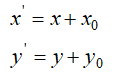我们对测试图进行水平和垂直正方向平移100像素，效果图如图Fig.1所示。

注意，黑色区域是我们默认填充的颜色，平移变换会出现图像跑到原图画布外面的情况，此时，原来的区域可以填充任意颜色，图像平移变换就这么简单。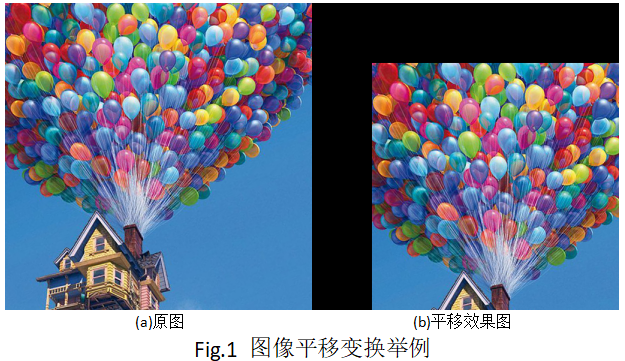[绘制与代码]

我们用C语言来实现图像平移变换算法，定义f_Transform.h文件，在文件中定义如下接口：

/*********************************************************
*Function:图像平移变换
*Params:
*          srcData:32bgra图像数据
*          width:图像宽度
*          height:图像高度
*          stride:图像幅度，对于32bgra格式而言，stride=width*4
*          xoffset：水平方向平移量
*          yoffset：垂直方向平移量
*Return:  0-成功，其他失败
*********************************************************/
int f_XYOfffset(unsigned char* srcData, int width, int height, int stride, int xoffset, int yofffset);

在这个接口中，定义了两个参数xoffset和yoffset分别用来表示水平和垂直的偏移向量，注意，如果xoffset和yoffset都为正数，则表示的是向水平和垂直的正方向偏移了xoffset和yoffset个像素距离，表现在结果图中，即图像向左偏移，反之，图像向右边偏移。

完整接口代码如下：

/*********************************************************
*Function:图像平移变换
*Params:
*          srcData:32bgra图像数据
*          width:图像宽度
*          height:图像高度
*          stride:图像幅度，对于32bgra格式而言，stride=width*4
*          xoffset：水平方向平移向量
*          yoffset：垂直方向平移向量
*Return:  0-成功，其他失败
*********************************************************/
int f_XYOfffset(unsigned char* srcData, int width, int height, int stride, int xoffset, int yoffset)
{
int ret = 0;
unsigned char* tempData = (unsigned char*)malloc(sizeof(unsigned char) * height * stride);
memcpy(tempData, srcData, sizeof(unsigned char) * height * stride);
unsigned char* pSrc = srcData;
for(int j = 0; j < height; j++)
{
for(int i = 0; i < width; i++)
{
int cx = i + xoffset;
int cy = j + yoffset;
if(cx >= 0 && cx < width && cy >= 0 && cy < height)
{
int pos = cx * 4 + cy * stride;
pSrc = tempData[pos];
pSrc = tempData[pos + 1];
pSrc = tempData[pos + 2];
}
else
{
pSrc = 0;
pSrc = 0;
pSrc = 0;
}
pSrc += 4;
}
}
free(tempData);
return ret;
};

接口到这里就写完了，仅仅30行左右，下面我们来写个测试代码：

#include "stdafx.h"
#include"imgRW\f_SF_ImgBase_RW.h"
#include"f_Transform.h"
int _tmain(int argc, _TCHAR* argv[])
{
//定义输入图像路径
char* inputImgPath = "Test.png";
//定义输出图像路径
char* outputImgPath = "res_offset.jpg";
//定义图像宽高信息
int width = 0, height = 0, component = 0, stride = 0;
//图像读取(得到32位bgra格式图像数据)
stride = width * 4;
int ret = 0;
//其他图像处理操作(这里以32位彩色图像灰度化为例)
//////////////////////////IMAGE PROCESS/////////////////////////////
//图像平移
int xoffset = -100;//图像向右平移
int yoffset = -100;//图像向左平移
//调用图像平移变换接口
ret = f_XYOfffset(bgraData, width, height, stride,xoffset, yoffset);
//保存平移变换结果图
ret = Trent_ImgBase_ImageSave(outputImgPath, width, height, bgraData, JPG);
printf("f_XYOffset is finished!");
////////////////////////////////////////////////////////////////////
}
return 0;

可以看到，在这段代码中，我们使用的都是C语言标准库，对于初学者而言，非常方便，通俗易懂。

图像缩放变换

[定义与算法]

图像缩放即图像缩小与放大，是图像处理中最常用的操作，可以说图像处理离不开图像缩放，好的图像缩放算法可以高清还原图像信息，对于各种复杂的图像应用而言意义重大。

假设图像中任意一点(x,y)，按照水平方向缩放比例a和垂直方向缩放比例b进行缩放，则缩放后点坐标(x’,y’)的计算如下：

当a和b小于1时，表现为缩小，大于1时表现为图像放大，等于1时不缩放；既然有了放大与缩小，就存在图像信息的删除与填充，如何进行精确计算缩放后的坐标位置？这里引入一个必需要讲解的内容---图像插值算法。

图像插值算法有很多，从最邻近插值，到二次插值、三次插值、卷积插值等等以及超分辨率算法等高级插值，可以说，图像插值是一门学问，单独成书的也很多很多。这里，我们讲解两种最常用也是最基础的插值算法：最邻近插值和双线性二次插值。

最邻近插值不明思义就是用距离它最近的点来代替它。如下图Fig.2所示，有A和B两个像素点，A的值为100，B的值为20，要计算AB之间的C点像素值，其中，C点距离A的距离为0.4，距离B的距离为0.6，那么，C点距离A点像素最近，则C=100，这就是最邻近插值。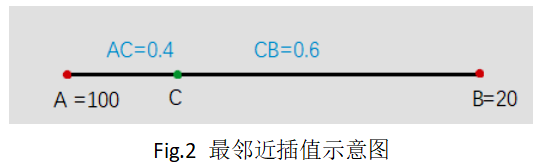最邻近插值计算量最小，但效果较差，往往会出现锯齿问题，即缩放后的图像边缘会出现锯齿毛刺，如图Fig.3所示，左边为原图，右边为最邻近插值放大两倍的结果，可以看到字母的边缘出现了锯齿状，非常不平滑。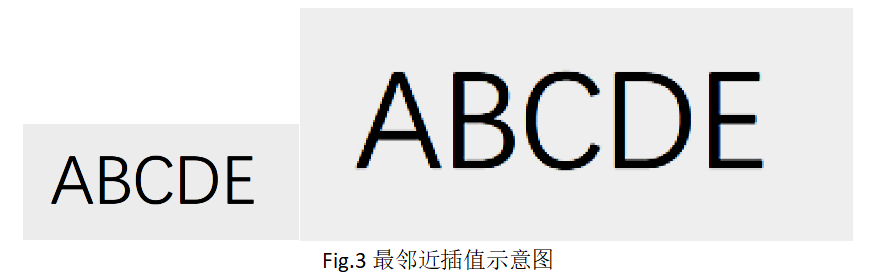双线性二次插值也称为一阶插值，这个算法说复杂也复杂，说简单也简单，如果你要从数学上讲明白，那要从拉格朗日插值多项式说起。

拉格朗日插值：

对于给定的n+1个节点，x0,x1,..xn，如果能够找到n+1个多项式，满足如下条件：，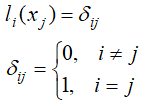那么，拉格朗日插值多项式P(x)表示如下：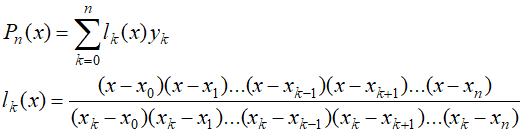其中，l(x)被称作插值基函数。

上述内容可以参考张铁所著《数值分析》136页部分，上述内容与二次插值有什么关系呢？这里我们一道例题来说明，该例题也来自张铁《数值分析》。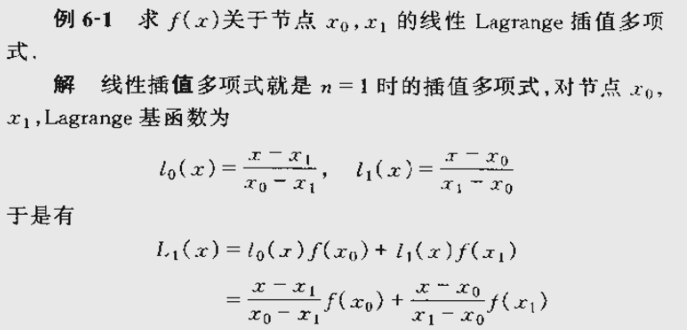对于x0和x1，它的一阶拉格朗日插值多项式L(x)求取如上所示，其中l0(x)和l1(x)分别表示对应权重，如果要计算x0到x1之间的任意一点x，那么计算L1(x)即可，看到这里我们明白，如果给定两个点，计算两点之间的任意一点插值，那么，我们只要可以使用上述1阶拉格朗日插值多项式即可，而这个方法就是二次插值的基础。

对于双线性二次插值，我们假设要计算的插值点(x,y)的值为f(x,y)，在它的附近有有f(i,j)，f(i+1,j)，f(i,j+1)和f(i+1,j+1)四个点，如下图Fig.4所示，f(x,y)的计算方法如下：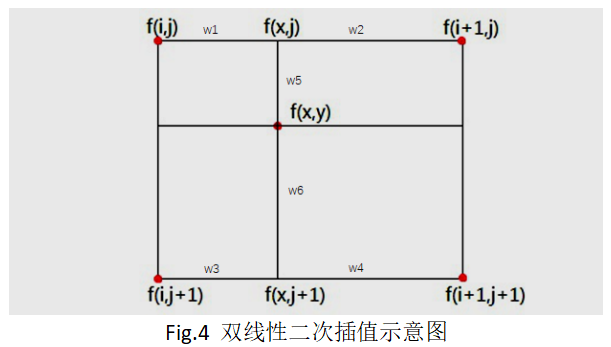①我们使用一阶拉格朗日插值计算点(i,j)和(i+1,j)之间的点(x,j)处的插值f(x,j)；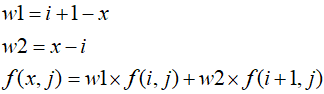②我们使用一阶拉格朗日插值计算点(i,j+1)和(i+1,j+1)之间的点(x,j+1)处的插值f(x,j+1)；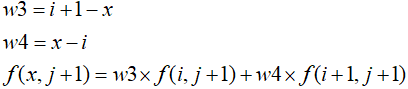③我们使用一阶拉格朗日插值计算(x,j)和(x,j+1)之间点(x,y)处的插值f(x,y)；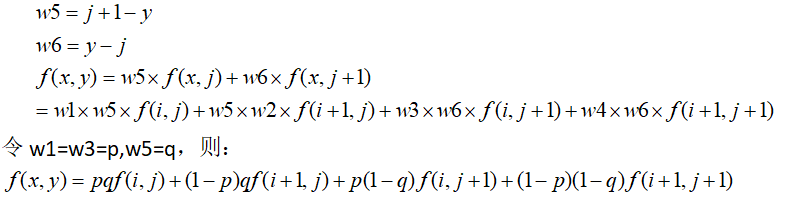这个公式就是双线性插值公式。我们用这个公式，对比最邻近插值效果，如下图Fig.5所示。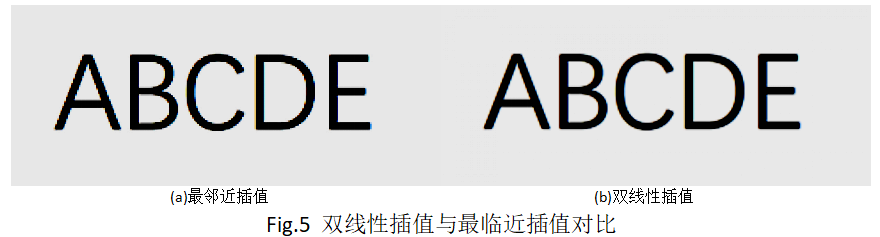[绘制与代码]

有了上述算法的解析，下面我们通过C语言来实现，首先定义接口如下：

/*********************************************************
*Function:图像缩放变换
*Params:
*          srcData:32bgra图像数据
*          width:图像宽度
*          height:图像高度
*          stride:图像幅度，对于32bgra格式而言，stride=width*4
*          scaleX:水平方向缩放比例,[0,]
*          scaleY:垂直方向缩放比例,[0,]
*          outW:缩放结果图宽度
*          outH:缩放结果图高度
*          outStride:缩放结果图Stride
*          interpolation：插值方式，0-最邻近插值，1-双线性插值
*Return:  缩放图像bgra数据指针
*********************************************************/
unsigned char* f_Zoom(unsigned char* srcData, int width, int height, int stride, float scaleX, float scaleY, int* outW, int* outH, int * outStride, int interpolation);

我们定义了f_Zoom的接口，这个接口中，由于缩放会改变图像大小，因此，我们返回一个缩放后的图像数据指针，同时，返回缩放后的图像宽高信息outW、outH和outStride；由于缩放包含水平和垂直方向的缩放因子，所以，添加水平缩放参数scaleX和垂直缩放参数scaleY，当scaleX小于1时表示水平缩小，等于1表示水平不缩放，大于1表示水平放大，垂直方向亦如此；最后，由于我们可以使用最邻近插值和双线性插值两种方式进行缩放，因此，添加了interpolation插值参数；

有了接口，我们给出完整的接口实现代码如下：

/*********************************************************
*Function:图像缩放变换
*Params:
*          srcData:32bgra图像数据
*          width:图像宽度
*          height:图像高度
*          stride:图像幅度，对于32bgra格式而言，stride=width*4
*          scaleX:水平方向缩放比例,[0,]
*          scaleY:垂直方向缩放比例,[0,]
*          outW:缩放结果图宽度
*          outH:缩放结果图高度
*          outStride:缩放结果图Stride
*          interpolation：插值方式，0-最邻近插值，1-双线性插值
*Return:  缩放图像bgra数据指针
*********************************************************/
unsigned char* f_Zoom(unsigned char* srcData, int width, int height, int stride, float scaleX, float scaleY, int* outW, int* outH, int * outStride, int interpolation)
{
int w = width * scaleX;
int h = height * scaleY;
int s = w * 4;
unsigned char* tempData = (unsigned char*)malloc(sizeof(unsigned char) * s * h);
memset(tempData, 255, sizeof(unsigned char) * s * h);
unsigned char* pTemp = tempData;
//最邻近插值
if(interpolation == 0)
{
for(int j = 0; j < h; j++)
{
for(int i = 0; i < w; i++)
{
int cx = CLIP3(i * width / w, 0, width - 1);
int cy = CLIP3(j * height / h, 0, height - 1);
int pos = cx * 4 + cy * stride;
pTemp = srcData[pos];
pTemp = srcData[pos + 1];
pTemp = srcData[pos + 2];
pTemp = srcData[pos + 3];
pTemp += 4;
}
}
}
else//双线性插值
{
for(int j = 0; j < h; j++)
{
for(int i = 0; i < w; i++)
{
float cx = CLIP3((float)i * width / w, 1, width - 2);
float cy = CLIP3((float)j * height / h, 1, height - 2);
int tx = (int)cx;
int ty = (int)cy;
float p = abs(cx - tx);
float q = abs(cy - ty);
int pos = tx * 4 + ty * stride;
int p1 = pos;
int p2 = pos + stride;
int p3 = pos + 4;
int p4 = pos + 4 + stride;
float a = (1.0f - p) * (1.0f - q);
float b = (1.0f - p) * q;
float c = p * (1.0f - q);
float d = p * q;
pTemp = CLIP3((a * srcData[p1 + 0] + b * srcData[p2 + 0] + c * srcData[p3 + 0] + d * srcData[p4 + 0]), 0, 255);
pTemp = CLIP3((a * srcData[p1 + 1] + b * srcData[p2 + 1] + c * srcData[p3 + 1] + d * srcData[p4 + 1]), 0, 255);
pTemp = CLIP3((a * srcData[p1 + 2] + b * srcData[p2 + 2] + c * srcData[p3 + 2] + d * srcData[p4 + 2]), 0, 255);
pTemp = CLIP3((a * srcData[p1 + 3] + b * srcData[p2 + 3] + c * srcData[p3 + 3] + d * srcData[p4 + 3]), 0, 255);
pTemp += 4;
}
}
}
*outW = w;
*outH = h;
*outStride = s;
return tempData;
};

代码不足60行，通俗易懂，最后给出接口的调用代码：

#include "stdafx.h"
#include"imgRW\f_SF_ImgBase_RW.h"
#include"f_Transform.h"
int _tmain(int argc, _TCHAR* argv[])
{
//定义输入图像路径
char* inputImgPath = "t150.png";
//定义图像宽高信息
int width = 0, height = 0, component = 0, stride = 0;
//图像读取(得到32位bgra格式图像数据)
stride = width * 4;
int ret = 0;
//其他图像处理操作(这里以32位彩色图像为例)
//////////////////////////IMAGE PROCESS/////////////////////////////
//图像缩放
int outW, outH, outS;
float scaleX = 5;
float scaleY = 5;
int interpolation = INTERPOLATE_BILINEAR;//INTERPOLATE_NEAREST;
char* outZoomImgPath = "res_zoom.png";
unsigned char* pResZoom = f_Zoom(bgraData, width, height, stride, scaleX, scaleY, &outW, &outH, &outS, interpolation);
ret = Trent_ImgBase_ImageSave(outZoomImgPath, outW, outH, pResZoom, PNG);
free(pResZoom);
printf("f_zoom is finished!");
////////////////////////////////////////////////////////////////////
return 0;
}

效果测试如下图Fig.6所示：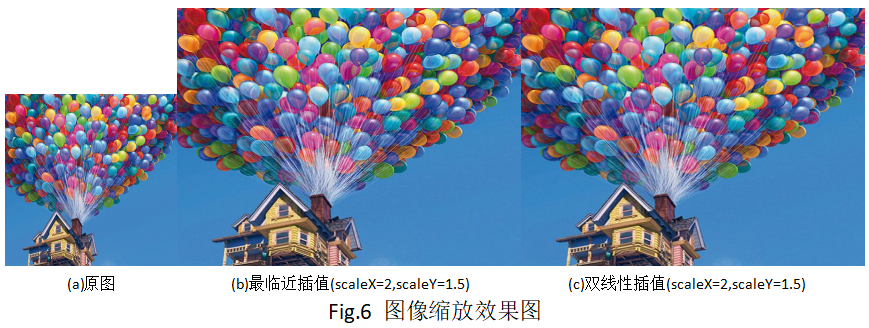图像旋转变换

[定义与算法]

图像旋转即将图像按照某个原点顺时针或者逆时针旋转某个角度。

如果平面上所有点(x,y)绕原点O旋转角度，旋转后的点为(x’,y’)，则两者正向和逆向计算公式如下：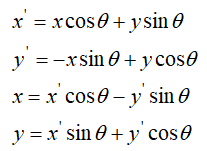公式的推导我们以正向变换为例，在极坐标系中，假设(x,y)到原点O距离为r，注意，这里O点表示上文平移后的图像中心点，(x,y)和原点的连线与x轴方向的夹角为b，旋转角度为a，旋转后坐标为(x’,y’)，如下图Fig.7所示，则按照极坐标公式有：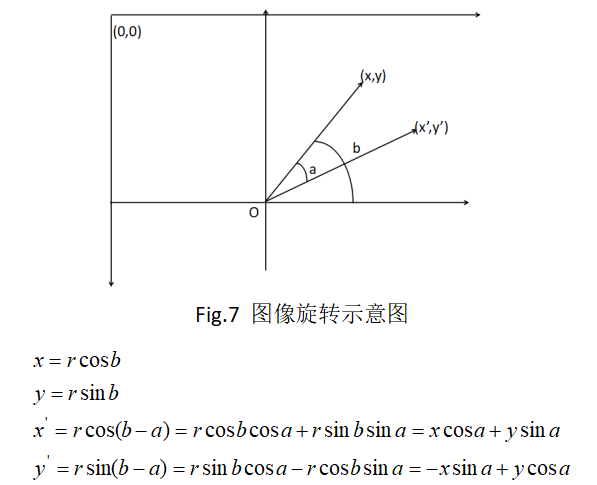上述便是正向推导过程，即由(x,y)到旋转后的点(x’,y’)。

在实际中，图像平面中的原点一般为左上角，也就是左上角为(0,0)点，垂直方向向下为正方向，与正常的笛卡尔坐标系不同。我们想要的往往是图像围绕图像中心点进行角度旋转，这个时候，我们需要把点(x,y)先转换为以图像中心为原点的坐标，也就是进行一定的坐标平移，然后再进行旋转变换。同时，为例避免孔洞现象，我们一般在计算的过程中，是按照逆向变换，根据目标图像素位置(x’,y’)计算它在原图中的位置(x,y)，一次完成旋转变换的。

假设旋转后图像的宽为W’，高为H’，点(x’,y’)映射到原图中的坐标为(x,y)，则计算过程如下：

①按照平移逆变换将(x’,y’)进行平移：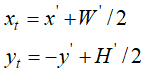②按照旋转逆变换公式将(x,y)进行变换：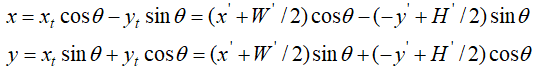③将坐标原点由图像中心平移至左上角：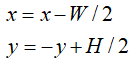④根据(x,y)位置选择插值算法插值得到最终旋转后的像素值；

上面的过程就是完整的图像旋转变换，下面我们将动手实践一下。

[绘制与代码]

我们首先定义一个图像旋转的接口，如下：

/*********************************************************
*Function:图像旋转变换
*Params:
*          srcData:32bgra图像数据
*          width:图像宽度
*          height:图像高度
*          stride:图像幅度，对于32bgra格式而言，stride=width*4
*          angle:图像旋转角度
*          outW:旋转结果图宽度
*          outH:旋转结果图高度
*          outStride:旋转结果图Stride
*          interpolation：插值方式，0-最邻近插值，1-双线性插值
*Return:  旋转图像bgra数据指针
*********************************************************/
unsigned char* f_Rotate(unsigned char* srcData, int width, int height, int stride, int angle, int* outW, int* outH, int* outStride, int interpolation);

图像旋转变换中，图像的大小发生了变化，因此这里我们的接口返回一个变换后的图像数据指针，与缩放接口类似，添加新图像宽高输出参数outW，outH和outStride；由于旋转变换需要角度信息，因此这里添加了角度输入参数angle，范围为0到360度，同时，设置插值算法参数interpolation；

按照前文的旋转变换算法公式，我们给出C代码如下：

/*********************************************************
*Function:图像旋转变换
*Params:
*          srcData:32bgra图像数据
*          width:图像宽度
*          height:图像高度
*          stride:图像幅度，对于32bgra格式而言，stride=width*4
*          angle:图像旋转角度
*          outW:旋转结果图宽度
*          outH:旋转结果图高度
*          outStride:旋转结果图Stride
*          interpolation：插值方式，0-最邻近插值，1-双线性插值
*Return:  旋转图像bgra数据指针
*********************************************************/
unsigned char* f_Rotate(unsigned char* srcData, int width, int height, int stride, int angle, int* outW, int* outH, int* outStride, int interpolation)
{
float degree = angle * PI / 180.0f;
float cx = 0, cy = 0, Cos = 0, Sin = 0;
Cos = cos(degree);
Sin = sin(degree);
//计算新图像的宽高
int w = width * Cos + height * Sin;
int h = height * Cos + width * Sin;
int s = w * 4;
*outW = w;
*outH = h;
*outStride = s;
//常量计算，用来优化速度
cx = -w / 2.0f * Cos - h / 2.0f * Sin + width / 2.0f;
cy = w / 2.0f * Sin - h / 2.0f * Cos + height / 2.0f;
unsigned char* tempData = (unsigned char*)malloc(sizeof(unsigned char) * s * h);
memset(tempData, 255, sizeof(unsigned char) * s * h);
unsigned char* pTemp = tempData;
//最邻近插值
if(interpolation == 0)
{
for(int j = 0; j < h; j++)
{
for(int i = 0; i < w; i++)
{
//这里实际上就是按照公式计算，进行了优化，把一些常量计算放到了外面的cx和cy中
int tx = i * Cos + j * Sin + cx;
int ty = j * Cos - i * Sin + cy;
if(tx >= 0 && tx < width && ty >= 0 && ty < height)
{
int pos = tx * 4 + ty * stride;
pTemp = srcData[pos];
pTemp = srcData[pos + 1];
pTemp = srcData[pos + 2];
pTemp = srcData[pos + 3];
}
else
{
pTemp = 0;
pTemp = 0;
pTemp = 0;
pTemp = 255;
}
pTemp += 4;
}
}
}
else//双线性插值
{
for(int j = 0; j < h; j++)
{
for(int i = 0; i < w; i++)
{
//这里实际上就是按照公式计算，进行了优化，把一些常量计算放到了外面的cx和cy中
float mx = i * Cos + j * Sin + cx;
float my = j * Cos - i * Sin + cy;
if(mx >= 0 && mx < width && my >= 0 && my < height)
{
int tx = (int)mx;
int ty = (int)my;
float p = abs(mx - tx);
float q = abs(my - ty);
int pos = tx * 4 + ty * stride;
int p1 = pos;
int p2 = pos + stride;
int p3 = pos + 4;
int p4 = pos + 4 + stride;
float a = (1.0f - p) * (1.0f - q);
float b = (1.0f - p) * q;
float c = p * (1.0f - q);
float d = p * q;
pTemp = CLIP3((a * srcData[p1 + 0] + b * srcData[p2 + 0] + c * srcData[p3 + 0] + d * srcData[p4 + 0]), 0, 255);
pTemp = CLIP3((a * srcData[p1 + 1] + b * srcData[p2 + 1] + c * srcData[p3 + 1] + d * srcData[p4 + 1]), 0, 255);
pTemp = CLIP3((a * srcData[p1 + 2] + b * srcData[p2 + 2] + c * srcData[p3 + 2] + d * srcData[p4 + 2]), 0, 255);
pTemp = CLIP3((a * srcData[p1 + 3] + b * srcData[p2 + 3] + c * srcData[p3 + 3] + d * srcData[p4 + 3]), 0, 255);
}
else
{
pTemp = 0;
pTemp = 0;
pTemp = 0;
pTemp = 255;
}
pTemp += 4;
}
}
}
return tempData;
};

本文的代码可以看到，基本都是将插值的interpolation条件判断放到了循环外面，导致代码段较长，实际上这样做是为了优化速度，增强代码可读性，大家可以体会一下。

我们对上面接口进行调用测试如下：

#include "stdafx.h"
#include"imgRW\f_SF_ImgBase_RW.h"
#include"f_Transform.h"
int _tmain(int argc, _TCHAR* argv[])
{
//定义输入图像路径
char* inputImgPath = "Test.png";
//定义图像宽高信息
int width = 0, height = 0, component = 0, stride = 0;
//图像读取(得到32位bgra格式图像数据)
stride = width * 4;
int ret = 0;
//其他图像处理操作(这里以32位彩色图像为例)
//////////////////////////IMAGE PROCESS/////////////////////////////
//图像旋转
int outW, outH, outS;
int angle = 80;
int interpolation = INTERPOLATE_NEAREST;//INTERPOLATE_BILINEAR;//INTERPOLATE_NEAREST;
char* outRotateImgPath = "res_rotate_nearest.jpg";
unsigned char* pResRotate = f_Rotate(bgraData, width, height, stride, angle, &outW, &outH, &outS, interpolation);
ret = Trent_ImgBase_ImageSave(outRotateImgPath, outW, outH, pResRotate, JPG);
free(pResRotate);
printf("f_Rotate is finished!");
////////////////////////////////////////////////////////////////////
return 0;
}

大家可以看到，调用非常方便，甚至比opencv更加通俗易懂。最后我们给出对应的测试效果，如图Fig.8所示。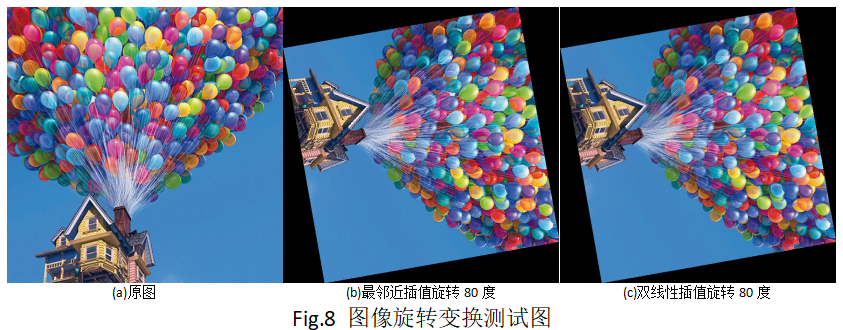本节完整的代码工程关注本人公众号“SF图像算法”有相关下载链接即可免费下载。

[知识扩展]

在本文中，我们详细了解了图像平移、图像缩放和图像旋转三种图像几何变换，也是最常用的图像基本变换。在这个过程中我们是单个一一讲解的，为的是让新手同学们能够各个击破，单独理解。而实际中，这三个变换可以通过一个仿射变换矩阵来统一表达，放这边还矩阵如下：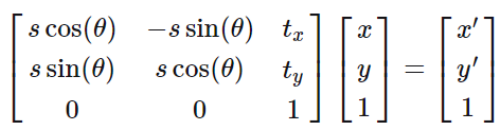其中，s表示缩放比例，tx和ty表示平移量，theta表示角度；

按照这个公式，我们可以一次计算出三种变换后对应的结果(x’,y’)，然后再进行插值计算即可，大家可以自己尝试一下；

对于图像变形和插值算法，多少年来，有无数相关的论文研究，近几年来，随着深度学习的飞速发展，基于卷积神经网络的超分辨率，图像复原以及图像变形的算法也是层出不穷，可见其意义之大！

最后，学海无涯，共勉！


展开全文图像旋转 图像缩放
• 新建一个图像，他的大小比原来的图像要大一些，因为平移图像位置发生变化，图像被移动后，会有一部分位置空出来。平移前和平移后的效果图如图所示： 【原图】 【平移后的图像】 原图和平移后的图像相比，很明显...
文章目录一、 平移1、思路2、实现代码二、缩放1、思路2、代码实现三、旋转变换1、思路
对文章内容如有异议欢迎在评论区提问
代码包：数字图像平移、旋转、缩放
一、 平移
1、思路
新建一个图像，他的大小比原来的图像要大一些，因为平移后图像位置发生变化，图像被移动后，会有一部分位置空出来。平移前和平移后的效果图如图所示：
【原图】【平移后的图像】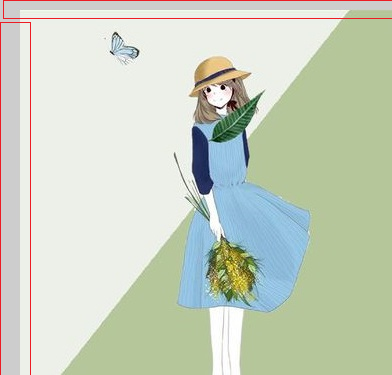原图和平移后的图像相比，很明显，红色框框住的那部分是平移后空出来的部分。
所以平移思路就是，将原先图像的横坐标和纵坐标分别加上一个数字，变成一个新的坐标，然后将原图中原坐标位置的RGB赋值给新图中新坐标。
2、实现代码
		//读原图
Mat image;

if (!image.data)
{
cout << "找不到图片或无法打开图片\n";
return -1;
}
namedWindow("image_source", CV_WINDOW_AUTOSIZE);
imshow("原图", image);

//--------------------平移变换--------------
int dx = 10, dy = 20;
Mat image_shift(image.rows + dx, image.cols+dy, CV_8UC3);//新建一个大于原图的图像，备用
//一个个像素进行处理
for (int counter1 = 0; counter1 < image.rows; counter1++)
{
for (int counter2 = 0; counter2 < image.cols; counter2++)
{
//将原坐标进行平移，并将原坐标上的RGB值赋给新坐标
image_shift.at<Vec3b>(counter1+dx, counter2+dy) = image.at<Vec3b>(counter1, counter2);
image_shift.at<Vec3b>(counter1+dx, counter2 + dy) = image.at<Vec3b>(counter1, counter2);
image_shift.at<Vec3b>(counter1+dx , counter2+ dy) = image.at<Vec3b>(counter1, counter2);
}
}
//imshow("平移", image_shift);
imwrite("1.jpg", image_shift);//将平移后的图像存储为名称为1.jpg的文件
//-------------- 平移完成-------------------

二、缩放
1、思路

先拿放大来说吧。把放大想象成这样一个过程，你画了一幅画，然后你拿了一个和这个画一样大的筛子（应该都有了解吧），放在画上，这样筛子的每个孔是不是对应画的非常小的一点区域。然后你就把筛子孔对应那个区域的RGB值赋给哪个筛子孔，然后你在把每一个筛子孔放大，就得到了放大后的图像。
就数字图像来说的话，比如你把图像要放大2倍，也就是说，新图像的像素数是原图像的2倍，但是你又必须把每个新图像的像素对应到某个原图像的像素上去，所以你就得将新图像的坐标乘以1/2，然后四射五入，得到原图像对应得像素，在RGB值对应起来。
上面其实和筛子原理是一样的，只不过计算机中不可能放大一个像素的大小，所以乘以1/2相当于是把筛子缩小，正好对应上画的大小。
效果图如下：是缩小效果图2、代码实现
//----------------尺度变换------------------
double mul_num = 0.8;//大于1是放大，小于1是缩小
int row_num = (int)floor(image.rows * mul_num), col_num = (int)floor(image.cols * mul_num);
Mat image_scale(row_num, col_num, CV_8UC3);
for (int counter1 = 0; counter1 < row_num; counter1++)
{
for (int counter2 = 0; counter2 < col_num; counter2++)
{
//将筛孔缩小，对上画的大小
int x = (int)1.0 / mul_num *counter1, y = (int)1.0 / mul_num *counter2;
image_scale.at<Vec3b>(counter1, counter2 ) = image.at<Vec3b>(x, y);
image_scale.at<Vec3b>(counter1, counter2 ) = image.at<Vec3b>(x, y);
image_scale.at<Vec3b>(counter1, counter2 ) = image.at<Vec3b>(x, y);
}
}
//imshow("尺度变换", image_scale);
imwrite("2.jpg", image_scale);//存储变换后的图像
//----------------尺度变换完成---------------

三、旋转变换
1、思路

如果直接按照仿射矩阵来旋转的话会出问题。因为那个是围绕左上角的原点来旋转的，你一旋转以后，他马上会越界访问，所以会出现问题。下面详细来说：如上图所示，将原图像按上述方式旋转以后，会超出原图像的区域。当然我们也有补救的办法，那就是创建一个相当大的新图像，不管它围绕原点怎么转，都不会出去的方案。但那个比较浪费。所以我用了下面的方法。
将它沿着它的中心旋转，这样就好控制新图像所在的范围了。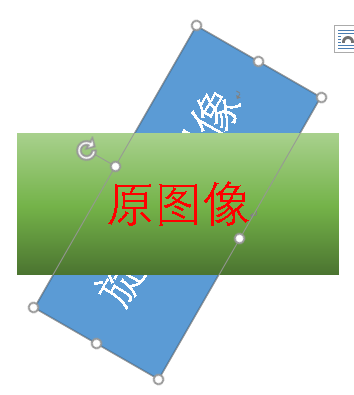这样就比较好了。
围绕中心旋转也比较好处理，我们还是回到前面的那个旋转结果啊。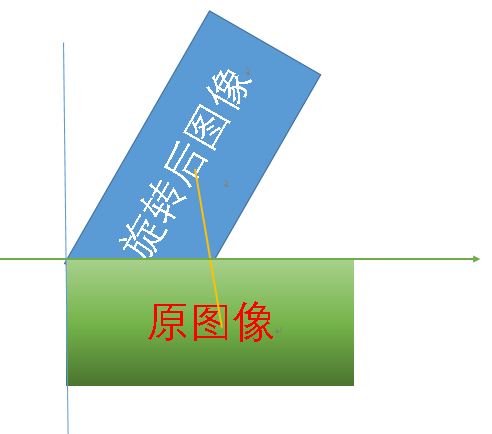把这两个图像放在一个坐标系中，可以看到，想要得到围绕中心旋转的图像，可以把上述旋转得到的图像的中心移动到原的图像的中心即可。也就是说观察新图像和原图像两者的中心得坐标发生了什么变化。
通过观察发现，新图像的中心横纵坐标分别减去，两中心间横纵坐标间的距离，就可以得到原图像中心坐标。如果对新图像的每个坐标都做上面的变化，那么就将它平移到了原图像中心的位置。
具体实现代码如下：

//----------------旋转变换------------------
double angle = 135.0 * 3.1415926 / 180.0;//把角度化成弧度
int dis = ceil(sqrt(pow(image.rows, 2) + pow(image.cols, 2)));//新图像的长宽应该是原图像的对角线长度
Mat image_nrota(dis, dis, CV_8UC3);

for (int counter1 = 0; counter1 < image.rows; counter1++)
{
for (int counter2 = 0; counter2 < image.cols; counter2++)
{
//计算应该平移的距离
float cenX = image_nrota.rows/2 - ((image.rows / 2) * cos(angle) - (image.cols/2) * sin(angle)),
cenY = image_nrota.cols/2 - ((image.rows / 2) * sin(angle) + (image.cols / 2) * cos(angle));
//按照仿射矩阵计算后进行平移
int x = counter1 * cos(angle) - counter2 * sin(angle) + cenX,
y = counter1 * sin(angle) + counter2 * cos(angle) + cenY;
image_nrota.at<Vec3b>(x, y) = image.at<Vec3b>(counter1, counter2);
image_nrota.at<Vec3b>(x, y) = image.at<Vec3b>(counter1, counter2);
image_nrota.at<Vec3b>(x, y) = image.at<Vec3b>(counter1, counter2);

}
}
//imshow("旋转", image_nrota);
imwrite("3.jpg", image_nrota);
//-----------旋转变换完成---------------


后面还有偏移变换，就不在赘述原理了，比较简单，直接上完整代码。

【change.h】
#pragma once
#include <opencv2/core/core.hpp>
#include <opencv2/highgui/highgui.hpp>
#include <iostream>
#include <cmath>
#include <Windows.h>

using namespace std;
using namespace cv;

//对图像进行平移操作
void shift(Mat &image, int dx, int dy)
{
Mat image_shift(image.rows + dx, image.cols + dy, CV_8UC3);//新建一个大于原图的图像，备用
//一个个像素进行处理
for (int counter1 = 0; counter1 < image.rows; counter1++)
{
for (int counter2 = 0; counter2 < image.cols; counter2++)
{
//将原坐标进行平移，并将原坐标上的RGB值赋给新坐标
image_shift.at<Vec3b>(counter1 + dx, counter2 + dy) = image.at<Vec3b>(counter1, counter2);
image_shift.at<Vec3b>(counter1 + dx, counter2 + dy) = image.at<Vec3b>(counter1, counter2);
image_shift.at<Vec3b>(counter1 + dx, counter2 + dy) = image.at<Vec3b>(counter1, counter2);
}
}
//imshow("平移", image_shift);
imwrite("1.jpg", image_shift);//将平移后的图像存储为名称为1.jpg的文件
}

//进行尺度变换
void scale(Mat &image, double mul_num)
{
int row_num = (int)floor(image.rows * mul_num), col_num = (int)floor(image.cols * mul_num);
Mat image_scale(row_num, col_num, CV_8UC3);
for (int counter1 = 0; counter1 < row_num; counter1++)
{
for (int counter2 = 0; counter2 < col_num; counter2++)
{
//将筛孔缩小，对上画的大小
int x = (int)1.0 / mul_num * counter1, y = (int)1.0 / mul_num * counter2;
image_scale.at<Vec3b>(counter1, counter2) = image.at<Vec3b>(x, y);
image_scale.at<Vec3b>(counter1, counter2) = image.at<Vec3b>(x, y);
image_scale.at<Vec3b>(counter1, counter2) = image.at<Vec3b>(x, y);
}
}
//imshow("尺度变换", image_scale);
imwrite("2.jpg", image_scale);//存储变换后的图像
}

//进行旋转
void rotation(Mat &image, double angle_in)
{
double angle = angle_in * 3.1415926 / 180.0;//把角度化成弧度
int dis = ceil(sqrt(pow(image.rows, 2) + pow(image.cols, 2)));//新图像的长宽应该是原图像的对角线长度
Mat image_nrota(dis, dis, CV_8UC3);

for (int counter1 = 0; counter1 < image.rows; counter1++)
{
for (int counter2 = 0; counter2 < image.cols; counter2++)
{
//计算应该平移的距离
float cenX = image_nrota.rows / 2 - ((image.rows / 2) * cos(angle) - (image.cols / 2) * sin(angle)),
cenY = image_nrota.cols / 2 - ((image.rows / 2) * sin(angle) + (image.cols / 2) * cos(angle));
//按照仿射矩阵计算后进行平移
int x = counter1 * cos(angle) - counter2 * sin(angle) + cenX,
y = counter1 * sin(angle) + counter2 * cos(angle) + cenY;
image_nrota.at<Vec3b>(x, y) = image.at<Vec3b>(counter1, counter2);
image_nrota.at<Vec3b>(x, y) = image.at<Vec3b>(counter1, counter2);
image_nrota.at<Vec3b>(x, y) = image.at<Vec3b>(counter1, counter2);

}
}
//imshow("旋转", image_nrota);
imwrite("3.jpg", image_nrota);
}

//垂直偏移变换
void voffset(Mat &image, double sv)
{
Mat image_voffset(sv*image.rows + image.cols, image.cols, CV_8UC3);
for (int counter1 = 0; counter1 < image.rows; counter1++)
{
for (int counter2 = 0; counter2 < image.cols; counter2++)
{
int x = counter1 * sv + counter2;

image_voffset.at<Vec3b>(x, counter2) = image.at<Vec3b>(counter1, counter2);
image_voffset.at<Vec3b>(x, counter2) = image.at<Vec3b>(counter1, counter2);
image_voffset.at<Vec3b>(x, counter2) = image.at<Vec3b>(counter1, counter2);

}
}
//imshow("垂直偏移", image_voffset);
imwrite("4.jpg", image_voffset);
}

//水平偏移变换
void hoffset(Mat &image, double sh)
{
Mat image_hoffset(image.rows, sh*image.rows + image.cols, CV_8UC3);
for (int counter1 = 0; counter1 < image.rows; counter1++)
{
for (int counter2 = 0; counter2 < image.cols; counter2++)
{
int y = counter1 * sh + counter2;

image_hoffset.at<Vec3b>(counter1, y) = image.at<Vec3b>(counter1, counter2);
image_hoffset.at<Vec3b>(counter1, y) = image.at<Vec3b>(counter1, counter2);
image_hoffset.at<Vec3b>(counter1, y) = image.at<Vec3b>(counter1, counter2);
}
}
//imshow("水平偏移", image_hoffset);
imwrite("5.jpg", image_hoffset);
}

【main.cpp】
#include <opencv2/core/core.hpp>
#include <opencv2/highgui/highgui.hpp>
#include <iostream>
#include <cmath>
#include <Windows.h>
#include "change.h"

using namespace std;
using namespace cv;
int main()
{
//读原图
Mat image;

if (!image.data)
{
cout << "找不到图片或无法打开图片\n";
return -1;
}
namedWindow("image_source", CV_WINDOW_AUTOSIZE);
imshow("原图", image);

//平移
shift(image, 10, 20);

//缩放
scale(image, 0.8);

//旋转
rotation(image, 45);

//垂直偏移
voffset(image, 1);

//水平偏移
hoffset(image, 1);
//连续播放变换后的图像
string picname = "";
Mat image2;
for (int counter = 1; counter < 6; counter++)
{
picname = '0'+ counter;
picname += ".jpg";
imshow("变换后的图像", image2);
waitKey(3000);
}

waitKey(0);
return 0;
}



展开全文• 本文主要讲述基于VC++6.0 MFC图像处理的应用知识，主要结合自己大三所学课程《数字图像处理》及课件进行讲解，主要通过MFC单文档视图实现显示BMP图片空间几何变换，包括图像平移、图形旋转、图像反转倒置镜像和图像...
       本文主要讲述基于VC++6.0 MFC图像处理的应用知识，主要结合自己大三所学课程《数字图像处理》及课件进行讲解，主要通过MFC单文档视图实现显示BMP图片空间几何变换，包括图像平移、图形旋转、图像反转倒置镜像和图像缩放的知识。同时文章比较详细基础，没有采用GDI+获取矩阵，而是通过读取BMP图片信息头和矩阵像素实现变换，希望该篇文章对你有所帮助，尤其是初学者和学习图像处理的学生。
【数字图像处理】一.MFC详解显示BMP格式图片
【数字图像处理】二.MFC单文档分割窗口显示图片
【数字图像处理】三.MFC实现图像灰度、采样和量化功能详解
【数字图像处理】四.MFC对话框绘制灰度直方图
【数字图像处理】五.MFC图像点运算之灰度线性变化、灰度非线性变化、阈值化和均衡化处理详解
免费资源下载地址：
一. 图像平移

前一篇文章讲述了图像点运算(基于像素的图像变换)，这篇文章讲述的是图像几何变换：在不改变图像内容的情况下对图像像素进行空间几何变换的处理方式。        点运算对单幅图像做处理，不改变像素的空间位置；代数运算对多幅图像做处理，也不改变像素的空间位置；几何运算对单幅图像做处理，改变像素的空间位置，几何运算包括两个独立的算法：空间变换算法和灰度级插值算法。
空间变换操作包括简单空间变换、多项式卷绕和几何校正、控制栅格插值和图像卷绕，这里主要讲述简单的空间变换，如图像平移、镜像、缩放和旋转。主要是通过线性代数中的齐次坐标变换。
图像平移坐标变换如下：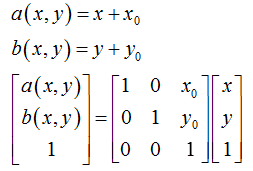运行效果如下图所示，其中BMP图片(0,0)像素点为左下角。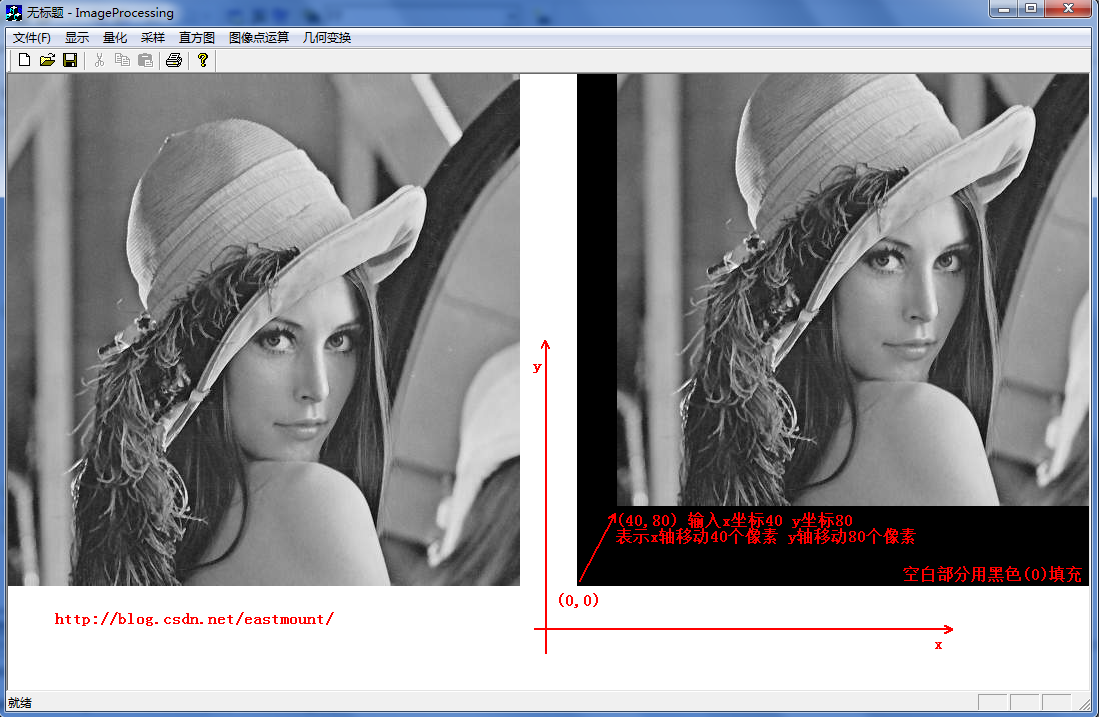其代码核心算法：
1.在对话框中输入平移坐标(x,y) m_xPY=x，m_yPY=y
2.定义Place=dlg.m_yPY*m_nWidth*3 表示当前m_yPY行需要填充为黑色
3.新建一个像素矩阵 ImageSize=new unsigned char[m_nImage]
4.循环整个像素矩阵处理
for(int i=0 ; i<m_nImage ; i++ ){
if(i<Place) {ImageSize[i]=black; continue;} //黑色填充底部 从小往上绘图                   else if(i>=Place && countWidth<dlg.m_xPY*3) {//黑色填充左部分                         ImageSize[i]=black; countWidth++;  continue;                   }                   else if(i>=Place && countWidth>=dlg.m_xPY*3) {//图像像素平移区域                        ImageSize[i]=m_pImage[m_pImagePlace];//原(0,0)像素赋值过去                        m_pImagePlace++; countWidth++;                        if(countWidth==m_nWidth*3) {
//一行填满 m_pImagePlace走到(0,1)                              number++; m_pImagePlace=number*m_nWidth*3;                        }                   }             }
5.写文件绘图fwrite(ImageSize,m_nImage,1,fpw)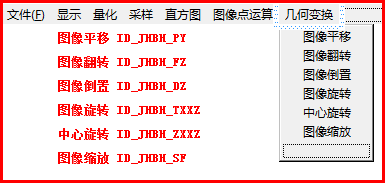第二步：设置平移对话框。将试图切换到ResourceView界面--选中Dialog,右键鼠标新建一个Dialog，并新建一个名为IDD_DIALOG_PY。编辑框(X)IDC_EDIT_PYX
和 (Y)IDC_EDIT_PYY，确定为默认按钮。设置成下图对话框：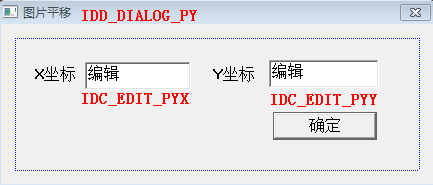第三步：在对话框资源模板空白区域双击鼠标—Create a new class创建一个新类--命名为CImagePYDlg。会自动生成它的.h和.cpp文件。打开类向导(Ctrl
W)，选择类名:CImagePYDlg添加成员变量如下图所示，同时在Message Maps中生成ID_JHBH_PY实现函数。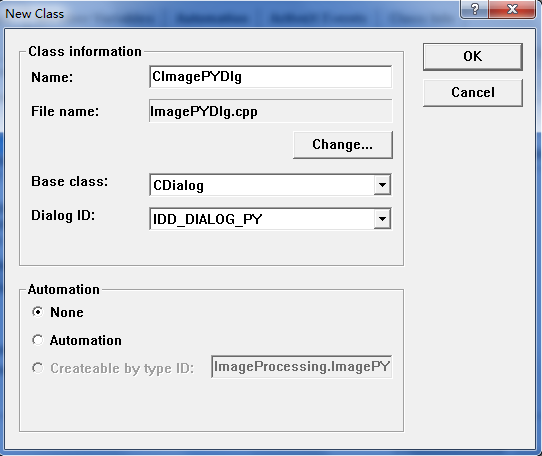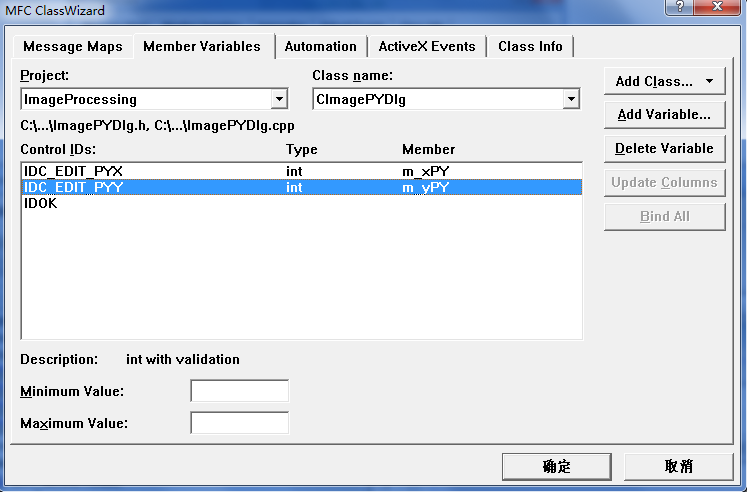第四步：在CImageProcessingView.cpp中添加头文件#include "ImagePYDlg.h"，并实现平移。/********************************************************/
/* 图像空间几何变换：图像平移 ID_JHBH_PY(几何变换-平移)
/* 使用平移对话框：CImagePYDlg dlg
/* 算法：f(x,y)=f(x+x0,y+y0)图像所有点平移，空的补黑'0'
/* 注意该图像平移方法只是从左上角(0,0)处开始平移
/* 其他方向原理相同 自己去实现
/********************************************************/

void CImageProcessingView::OnJhbhPy()
{
if(numPicture==0) {
AfxMessageBox("载入图片后才能空间平移!",MB_OK,0);
return;
}
//定义采样对话框也是用来空间变换平移的坐标
CImagePYDlg dlg;
if( dlg.DoModal()==IDOK ) //显示对话框
{
//采样坐标最初为图片的自身像素
if( dlg.m_xPY>m_nWidth || dlg.m_yPY>m_nHeight ) {
AfxMessageBox("图片平移不能为超过原图长宽!",MB_OK,0);
return;
}
AfxMessageBox("图片空间变换-平移!",MB_OK,0);

//打开临时的图片 读写文件
FILE *fpo = fopen(BmpName,"rb");
FILE *fpw = fopen(BmpNameLin,"wb+");

/************************************************************/
/* 图片空间变换-平移
/* 坐标(dlg.m_xPY,dlg.m_yPY)表示图像平移的坐标
/* 先用Plave计算出平移后的起始坐标，其他的坐标赋值为'0'黑色
/* 然后依次平移坐标，空的赋为黑色，否则填充
/************************************************************/

/******************************************************************/
/* 严重错误1：数组变量赋值相等
/* 在View.h中定义变量 BYTE *m_pImage 读入图片数据后的指针
/* 建立临时变量数组,让它平移变换 unsigned char *ImageSize
/* ImageSize=m_pImage(错误)
/* 会导致ImageSize赋值变换时m_pImage也产生了变换,所以输出全为黑色
/*     因为它俩指向了相同的数组地址
/* 解决方法：使用下面C++的new方法动态分配或for循环i=m_nImage赋值
/******************************************************************/

/*临时变量存储的像素与m_pImage相同，便于处理图像*/
unsigned char *ImageSize;
ImageSize=new unsigned char[m_nImage];  //new和delete有效的进行动态内存的分配和释放

int Place;                    //建立临时坐标 记录起始坐标(0,0)平移过来的位置
int m_pImagePlace;            //原始图像平移为(0,0) 图像把它平移到Place位置
unsigned char black;          //填充黑色='0'

/************************************************************/
/* for(int i=0 ; i<m_nHeight ; i++ )
/* for(int j=0 ; j<m_nWidth ; j++ )
/* 不能使用的上面的因为可能图像的最后一行没有完整的一行像素
/* 这样会出现exe报错,使用m_nImage读写所有像素比较正确
/************************************************************/

Place=dlg.m_yPY*m_nWidth*3;   //前m_yPY行都要填充为黑色
black=0;                       //颜色为黑色
m_pImagePlace=0;               //图像处事位置为(0,0),把该点像素平移过去
int countWidth=0;              //记录每行的像素个数,满行时变回0
int number=0;                  //数字记录使用的像素行数,平移时使用

for(int i=0 ; i<m_nImage ; i++ )
{
/*如果每行的像素填满时清为0*/
if(countWidth==m_nWidth*3) {
countWidth=0;
}

/*第一部分：到平移后像素位置前面的所有像素点赋值为黑色*/
if(i<Place) {
ImageSize[i]=black;     //赋值为黑色
continue;
}

/*第二部分：平移区域的左边部分赋值为黑色*/
else if(i>=Place && countWidth<dlg.m_xPY*3) { //RGB乘3
ImageSize[i]=black;     //赋值为黑色
countWidth++;
continue;
}

/****************************/
/* 各部分如图所示:
/* 000000000000000000000000
/* 000000000000000000000000
/* 0000000.................
/* 0000000.................
/* 0000000.................
/* 0000000.................
/* 点表示像素部分,0为黑色
/****************************/

/* 重点错误提示：由于bmp图像显示是从左下角开始存储(0,0)点所以输出图像为 */
/* bmp图像是从左下角到右上角排列的 */

/****************************/
/* 各部分如图所示:
/* 0000000.................
/* 0000000.................
/* 0000000.................
/* 0000000.................
/* 000000000000000000000000
/* 000000000000000000000000
/* 点表示像素部分,0为黑色
/****************************/

/*第三部分：图像像素平移区域*/
else if(i>=Place && countWidth>=dlg.m_xPY*3)
{
ImageSize[i]=m_pImage[m_pImagePlace];
m_pImagePlace++;
countWidth++;
if(countWidth==m_nWidth*3)
{
number++;
m_pImagePlace=number*m_nWidth*3;
}
}
}

fwrite(ImageSize,m_nImage,1,fpw);
fclose(fpo);
fclose(fpw);
numPicture = 2;
level=200;        //200表示几何变换
Invalidate();
}
}        同时在ShowBitmap中添加level标记重新绘制图片，代码如下：else        //图像几何变换
if(level=200)
{
}       运行时需要注意一点：BMP图像在处理过程中可能会出现一些斜线，而平移(40,60)位移量时可能出现如下。他是因为BMP格式有个非常重要的规定，要求每一扫描的字节数据必须能被4整除，也就是Dword对齐(长度4字节)，如果图像的一行字节数不能被4整除，就需要在每行末尾不起0达到标准。
例如一行像素为97字节，我们就需要补3个字节吗，数值可以是0，但是我们在BMP格式的信息头里说明了其宽度，所以补齐后对我们没有影响，所以后面补若干个字节的0即可直到被4整除。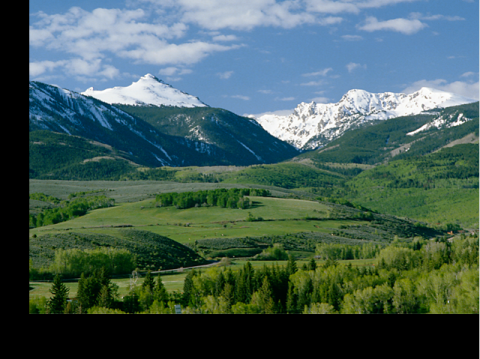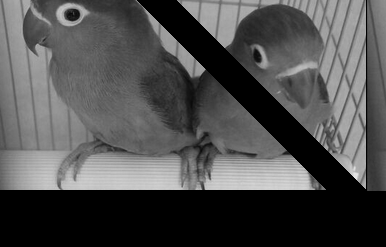通过后面的图像缩放后，我从学做了一遍这个补齐的缩放。代码如下，能够实现完美平移。nice啊~void CImageProcessingView::OnJhbhPy()
{
if(numPicture==0) {
AfxMessageBox("载入图片后才能空间平移!",MB_OK,0);
return;
}
//定义采样对话框也是用来空间变换平移的坐标
CImagePYDlg dlg;
if( dlg.DoModal()==IDOK ) //显示对话框
{
//采样坐标最初为图片的自身像素
if( dlg.m_xPY>m_nWidth || dlg.m_yPY>m_nHeight ) {
AfxMessageBox("图片平移不能为超过原图长宽!",MB_OK,0);
return;
}
AfxMessageBox("图片空间变换-平移!",MB_OK,0);

//打开临时的图片 读写文件
FILE *fpo = fopen(BmpName,"rb");
FILE *fpw = fopen(BmpNameLin,"wb+");

int num;            //记录每行多余的图像素数个数
int sfSize;         //补齐后的图像大小
//重点:图像的每行像素都必须是4的倍数：1*1的图像为 r g b 00H
if(m_nWidth*3%4!=0)
{
num=(4-m_nWidth*3%4);
sfSize=(m_nWidth*3+num)*m_nHeight; //每行多number个
}
else
{
num=0;
sfSize=m_nWidth*m_nHeight*3;
}
//注意:假如最后一行像素不足,我默认处理为完整的一行,不足补00H
//总之处理后的图像总是m*n且为4倍数,每行都完整存在

/*更改文件头信息 定义临时文件头结构变量*/
bfhsf=bfh;
bihsf=bih;
bfhsf.bfSize=sfSize+54;

CString str;
str.Format("补齐=%d",num);
AfxMessageBox(str);

/*临时变量存储的像素与sfSize相同 new和delete有效的进行动态内存的分配和释放*/
unsigned char *ImageSize;
ImageSize=new unsigned char[sfSize];

int Place;                    //建立临时坐标 记录起始坐标(0,0)平移过来的位置
int m_pImagePlace;            //原始图像平移为(0,0) 图像把它平移到Place位置
unsigned char black=0;        //填充黑色='0'
unsigned char other=0;        //补码00H='\0'

Place=dlg.m_yPY*(m_nWidth*3+num); //前m_yPY行都要填充为黑色
m_pImagePlace=0;                  //图像处事位置为(0,0),把该点像素平移过去
int countWidth=0;                 //记录每行的像素个数,满行时变回0
int number=0;                     //数字记录使用的像素行数,平移时使用

for(int i=0 ; i<sfSize ; i++ )
{
/*第一部分：到平移后像素位置前面的所有像素点赋值为黑色*/
if(i<Place)
{
ImageSize[i]=black;     //赋值为黑色
continue;
}

/*第二部分：平移区域的左边部分赋值为黑色*/
else if(i>=Place && countWidth<dlg.m_xPY*3)  //RGB乘3
{
ImageSize[i]=black;     //赋值为黑色
countWidth++;
continue;
}

/*第三部分：图像像素平移区域*/
else if(i>=Place && countWidth>=dlg.m_xPY*3)
{
ImageSize[i]=m_pImage[m_pImagePlace];
m_pImagePlace++;
countWidth++;
if(countWidth==m_nWidth*3)
{
if(num==0)
{
countWidth=0;
number++;
m_pImagePlace=number*m_nWidth*3;
}
else //num为补0
{
for(int j=0;j<num;j++)
{
i++;
ImageSize[i]=other;
}
countWidth=0;
number++;
m_pImagePlace=number*(m_nWidth*3+num); //重点：添加Num
}
}
}
}

fwrite(ImageSize,sfSize,1,fpw);
fclose(fpo);
fclose(fpw);
numPicture = 2;
level=200;        //200表示几何变换
Invalidate();
}
}        运行效果如下图所示，完美平移，其他算法遇到斜线问题类似补齐即可。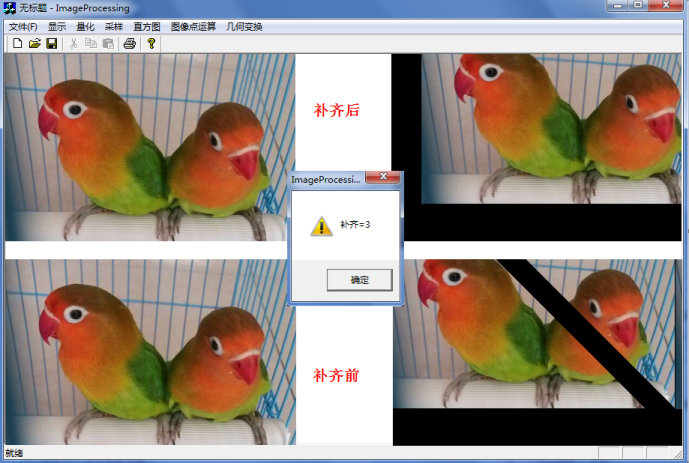二. 图像镜像
1.水平镜像翻转        其变换矩阵如下：                                 X=width-X0-1   (width为图像宽度)
Y=Y0        打开类向导，在CImageProcessingView中添加IDs为ID_JHBH_FZ，生成函数，代码如下：
/* 几何变换 图像翻转：自己对这个功能比较感兴趣,做个图像反转 */
void CImageProcessingView::OnJhbhFz()
{
if(numPicture==0) {
AfxMessageBox("载入图片后才能空间反转!",MB_OK,0);
return;
}
AfxMessageBox("图片空间变换-反转图像!",MB_OK,0);

//打开临时的图片
FILE *fpo = fopen(BmpName,"rb");
FILE *fpw = fopen(BmpNameLin,"wb+");

/*new和delete有效的进行动态内存的分配和释放*/
unsigned char *ImageSize;
ImageSize=new unsigned char[m_nImage];
int countWidth=0;        //记录每行的像素个数,满行时变回0
int Place;               //记录图像每行的位置,便于图像反转
int number=0;            //数字记录使用的像素行数
Place=m_nWidth*3-1;

//翻转矩阵: y=y0 x=width-x0-1
for(int i=0 ; i<m_nImage ; i++ )
{
if(countWidth==m_nWidth*3)
{
countWidth=0;
}
ImageSize[i]=m_pImage[Place]; //(0,0)赋值(0,width*3-1)像素
Place--;
countWidth++;
if(countWidth==m_nWidth*3)
{
number++;
Place=number*m_nWidth*3-1;
}
}

fwrite(ImageSize,m_nImage,1,fpw);
fclose(fpo);
fclose(fpw);
numPicture = 2;
level=200;
Invalidate();
}        运行效果如下图所示，其中还是存在一些小BUG，如前面的BMP图补0凑齐4整数倍宽度或颜色失帧。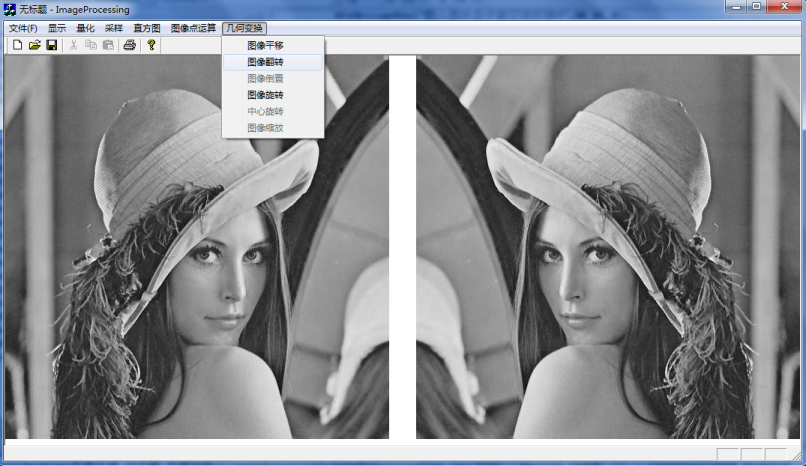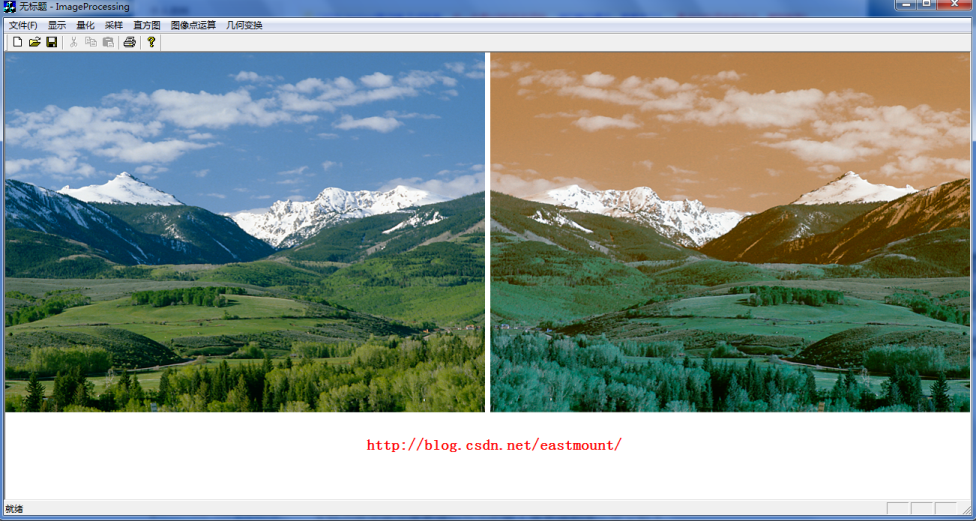2.垂直镜像倒转
其中变换矩阵如下：
X=X0
Y=height-Y0-1   (height为图像高度)        它相当于把原图的像素矩阵的最后一行像素值赋值给第一行，首先找到(0,0)对应的(height-1,0)像素值，然后依次赋值该行的像素数据；最后当前行赋值结束，依次下一行。重点是找到每行的第一个像素点即可。
代码中引用两个变量：Place=(m_nWidth*3)*(m_nHeight-1-1)即是(height-1,0)最后一行的第一个像素点；然后是循环中Place=(m_nWidth*3)*(m_nHeight-number-1)找到每行的第一个像素点。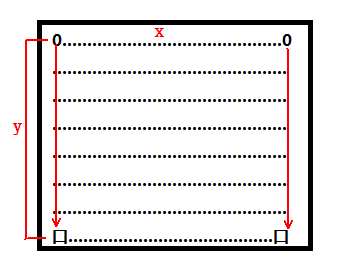同样通过类向导生成函数void CImageProcessingView::OnJhbhDz()，代码如下：/* 几何变换 图像倒转 */
void CImageProcessingView::OnJhbhDz()
{
if(numPicture==0) {
AfxMessageBox("载入图片后才能空间反转!",MB_OK,0);
return;
}
AfxMessageBox("图片空间变换-反转图像!",MB_OK,0);

//打开临时的图片
FILE *fpo = fopen(BmpName,"rb");
FILE *fpw = fopen(BmpNameLin,"wb+");

/*new和delete有效的进行动态内存的分配和释放*/
unsigned char *ImageSize;
ImageSize=new unsigned char[m_nImage];
int countWidth=0;                   //记录每行像素个数,满行时变回0
int Place;                          //每列位置
int number=0;                       //像素行数
Place=(m_nWidth*3)*(m_nHeight-1-1); //0行存储

//翻转矩阵: x=x0 y=height-y0-1
for(int i=0 ; i<m_nImage ; i++ )
{
ImageSize[i]=m_pImage[Place]; //(0,0)赋值(0,0)像素
Place++;
countWidth++;
if(countWidth==m_nWidth*3)
{
countWidth=0;
number++;
Place=(m_nWidth*3)*(m_nHeight-number-1);
}
}

fwrite(ImageSize,m_nImage,1,fpw);
fclose(fpo);
fclose(fpw);
numPicture = 2;
level=200;
Invalidate();
}        运行结果如下图所示，第二张图颜色没有失帧或变灰，这完全可以怀疑在翻转过程中RGB像素编程BGR后导致的结果，最终实现了翻转图像，但灰度存在一定；所以如果改为RBG顺序不变化即可原图颜色显示。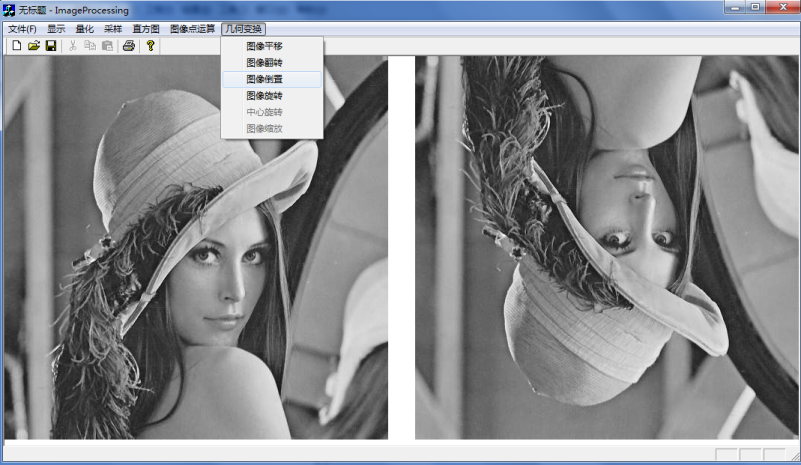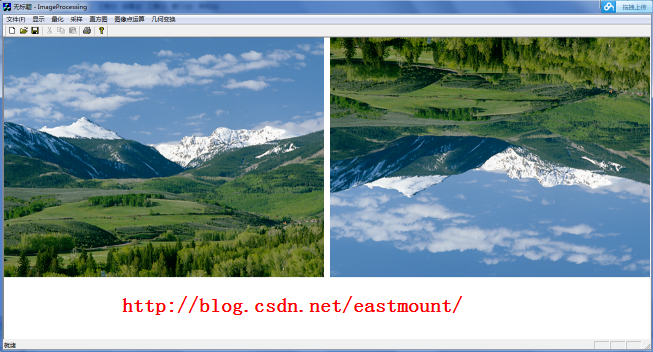三. 图像旋转
图像饶原点旋转顺时针theta角矩阵变换如下：注意BMP图像(0,0)左下角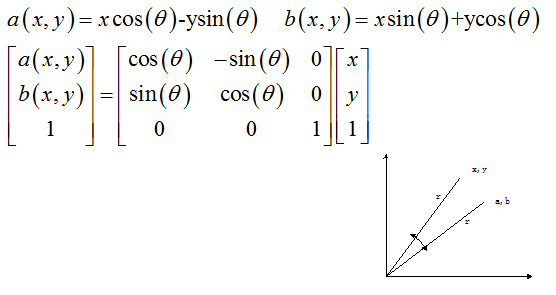写到这里真心觉得写底层的代码非常困难啊！尤其是以为像素转换二维像素，同时也觉得当时的自己算法部分还是很强大的，也感觉到如果采用GDI+操作像素矩阵Matrix或ColorMatrix是多么的方便，因为它定义好了X和Y向量，这就是为什么Android前面写的图像处理要容易得多。但是效率高~        好像利用GDI+旋转通过几句代码即可：
matrix.Rotate(15); //矩阵旋转15度
graph.SetTransform(&matrix);
graph.DrawImage(&image,points,3);
下面这部分代码是实现Android旋转的：参考我的博客//旋转图片
private void TurnPicture() {
Matrix matrix = new Matrix();
turnRotate=turnRotate+15;
//选择角度 饶(0,0)点选择 正数顺时针 负数逆时针 中心旋转
matrix.setRotate(turnRotate,bmp.getWidth()/2,bmp.getHeight()/2);
Bitmap createBmp = Bitmap.createBitmap(bmp.getWidth(), bmp.getHeight(), bmp.getConfig());
Canvas canvas = new Canvas(createBmp);
Paint paint = new Paint();
canvas.drawBitmap(bmp, matrix, paint);
imageCreate.setBackgroundColor(Color.RED);
imageCreate.setImageBitmap(createBmp);
textview2.setVisibility(View.VISIBLE);
}        实现效果如下图所示：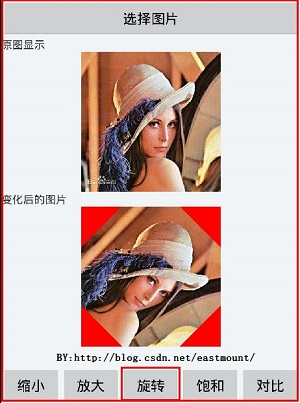言归正传，新建Dialog如下图所示，设置ID_DIALOG_XZ和变量：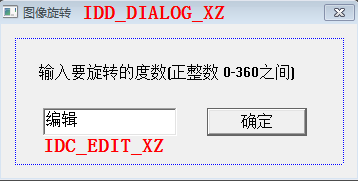再点击空白处创建CImageXZDlg类(旋转)，它会自动生成.h和.cpp文件。打开类向导生成CImageXZDlg类的成员变量m_xzds(旋转度数)，并设置其为int型(最大值360 最小值0)。
在类向导(Ctrl+W)选择类CImageProcessingView，为ID_JHBH_TXXZ(图像旋转)添加函数，同时添加头文件#include "ImageXZDlg.h"/**********************************************************/
/* 几何变换：图片旋转
/* 先添加对话框:IDD_JHBH_TXXZ(图像旋转),创建新类CImageXZDlg
/* 创建输入度数的:m_xzds Member variables 为int 0-360间
/**********************************************************/

void CImageProcessingView::OnJhbhTxxz()
{
if(numPicture==0) {
AfxMessageBox("载入图片后才能空间旋转!",MB_OK,0);
return;
}

//定义对话框并调用对话框
CImageXZDlg dlg;
if( dlg.DoModal()==IDOK ) //显示对话框
{
AfxMessageBox("图片空间变换-旋转图像!",MB_OK,0);
//读写文件
FILE *fpo = fopen(BmpName,"rb");
FILE *fpw = fopen(BmpNameLin,"wb+");

/*new和delete有效的进行动态内存的分配和释放*/
unsigned char *ImageSize;
ImageSize=new unsigned char[m_nImage];
int Place;          //记录图像每行的位置,便于图像旋转

/*定义PA=3.14时使用的方法是arcsin(1.0/2)*6即为π*/
double PA;
PA=asin(0.5)*6;

/*把输入的0-360的正整数度数转换为角度,30度=π/6*/
double degree;
degree=PA*dlg.m_xzds/180;   //调用dlg.m_xzds(旋转度数)

//对应的二维矩阵 注意图像矩阵从左下角开始处理 它最终要转换成一维存储
int X,Y;               //图像变换前通过一维矩阵转换为二维
int XPlace,YPlace;

//输出转换为的角度
CString str;
str.Format("转换后的角度=%f",degree);
AfxMessageBox(str);

//图像旋转处理
for(int i=0 ; i<m_nImage ; i++ )
{
//原图：一维矩阵转换为二维矩阵
X=(i/3)%m_nWidth;
Y=(i/3)/m_nWidth;
//注意错误：X=i/m_nHeight Y=i%m_nWidth; 只输出最后1/3

//图像旋转为：a(x,y)=x*cos-y*sin b(x,y)=x*sin+y*cos
XPlace=(int)(X*cos(degree)-Y*sin(degree));
YPlace=(int)(X*sin(degree)+Y*cos(degree));

//在转换为一维图想输出
if( (XPlace>=0 && XPlace<=m_nWidth) && (YPlace>=0 && YPlace<=m_nHeight) )
{
Place=YPlace*m_nWidth*3+XPlace*3;
//在图像范围内赋值为该像素
if(Place+2<m_nImage)
{
ImageSize[i]=m_pImage[Place];
i++;
ImageSize[i]=m_pImage[Place+1];
i++;
ImageSize[i]=m_pImage[Place+2];
}
//否则赋值为黑色
else
{
ImageSize[i]=0;
i++;
ImageSize[i]=0;
i++;
ImageSize[i]=0;
}
}
//否则赋值为黑色
else
{
ImageSize[i]=0;
i++;
ImageSize[i]=0;
i++;
ImageSize[i]=0;
}
}

fwrite(ImageSize,m_nImage,1,fpw);
fclose(fpo);
fclose(fpw);
numPicture = 2;
level=200;        //几何变换
Invalidate();
}
}        运行效果如下图所示，中心旋转太难了！找到中心那个位置就不太容易，我做不下去了，fuck~同时旋转过程中，由于是饶左下角(0,0)实现，故有的角度会到界面外显示全黑。下图分别旋转15度和355度。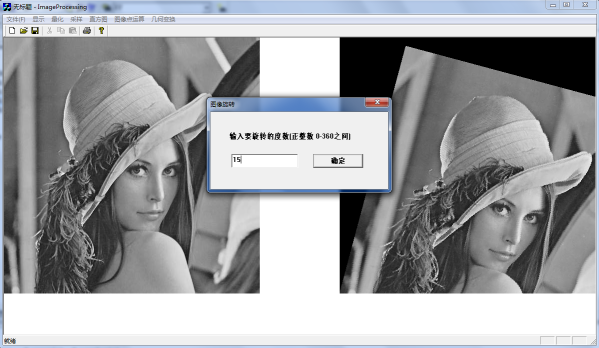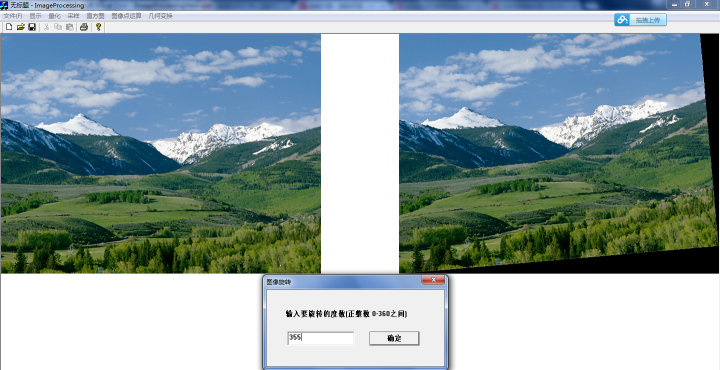四. 图像缩放
图像缩放主要有两种方法：
1.最近邻插值：向后映射时，输出图像的灰度等于离它所映射位置最近的输入图像的灰度值。其中向前映射和向后映射如下：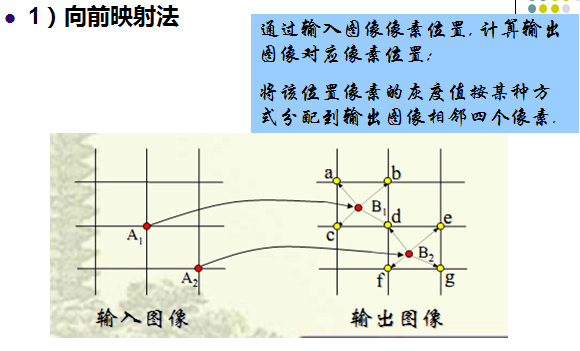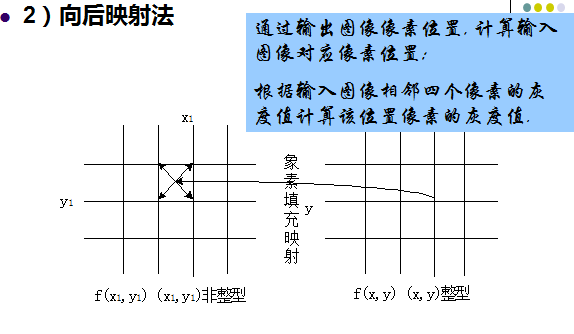对于向前映射每个输出图像的灰度要经过多次运算，对于向后映射，每个输出图像的灰度只经过一次运算。在实际应用中，更多的是采用向后映射法，其中根据四个相邻像素灰度值计算某个位置的像素灰度值即为灰度级插值。
2.双线性插值：四点确定一个平面函数，属于过约束问题。即单位正方形顶点已知，求正方形内任一点的f(x,y)值。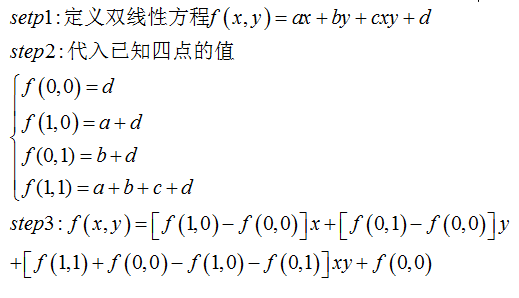换个通熟的说法，如下图所示。采用最近邻插值法就是P(x,y)像素值采用四舍五入等于离它最近的输入图像像素值。分别计算它到四个顶点之间的距离，但是这样会造成图像的马赛克、锯齿等现象。而采用双线性插值法，主要通过该坐标周围的四个像素值，按照比例混合计算器近似值。比例混合的依据是离哪个像素近，哪个像素的比例越大。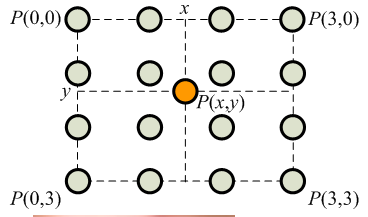下面是采用最近邻插值法的过程，注意BMP图缩放还需修改头文件信息。
第一步：在资源视图中添加“图像缩放”Dialog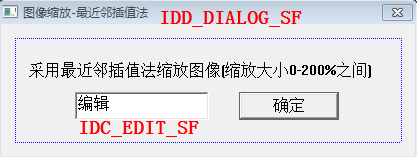第二步：点击空白处创建对话框的类CImageSFDlg，同时打开类向导为其添加成员变量m_sfbs(缩放倍数)，其为int型在0-200之间。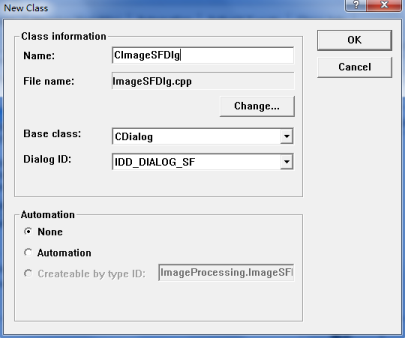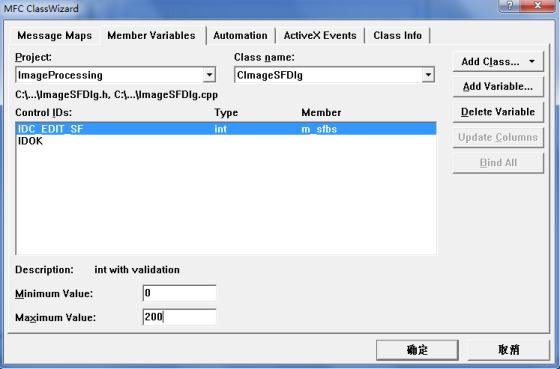第三步：打开类向导为其添加成员函数void CImageProcessingView::OnJhbhSf() 并实现缩放。同时添加头文件#include "ImageSFDlg.h"。/*******************************************************************/
/* ID_JHBH_SF: 几何运算-缩放-最近邻插值算法
/* 算法思想:输出图像的灰度等于离它所映射位置最近的输入图像的灰度值
/* 先计算出放大缩小后的长宽，根据它计算找原图中的点灰度，四舍五入
/*******************************************************************/

void CImageProcessingView::OnJhbhSf()
{
if(numPicture==0) {
AfxMessageBox("载入图片后才能几何缩放图像!",MB_OK,0);
return;
}

CImageSFDlg dlg;           //定义缩放对话框
if( dlg.DoModal()==IDOK )
{
//采样坐标最初为图片的自身像素  m_sfbs(缩放倍数)
if( dlg.m_sfbs==0 ) {
AfxMessageBox("输入图片缩放倍数不能为0!",MB_OK,0);
return;
}

FILE *fpo = fopen(BmpName,"rb");
FILE *fpw = fopen(BmpNameLin,"wb+");

/*先求缩放后的长宽*/
int sfWidth,sfHeight;                            //缩放后的长宽
int sfSize;                                      //缩放后的图像大小
sfWidth=(int)(m_nWidth*(dlg.m_sfbs*1.0)/100);    //24位图像RGB必须是3倍数 循环读取时为RGB
sfHeight=(int)(m_nHeight*(dlg.m_sfbs*1.0)/100);
int number;                                      //记录每行多余的图像素数个数

//重点:图像的每行像素都必须是4的倍数：1*1的图像为 r g b 00H
if(sfWidth*3%4!=0) {
number=(4-sfWidth*3%4);
sfSize=(sfWidth*3+(4-sfWidth*3%4))*sfHeight;
}
else {
number=0;
sfSize=sfWidth*sfHeight*3;
}
//注意:假如最后一行像素不足,我默认处理为完整的一行,不足补00H
//总之处理后的图像总是m*n且为4倍数,每行都完整存在

/*更改文件头信息 定义临时文件头结构变量*/
bfhsf=bfh;
bihsf=bih;

bfhsf.bfSize=sfSize+54;
bihsf.biWidth=sfWidth;
bihsf.biHeight=sfHeight;

//显示部分m_nDrawWidth<650显示原图,否则显示
flagSF=1;                         //图像缩放为1标识变量
m_nDrawWidthSF=sfWidth;
m_nDrawHeightSF=sfHeight;

unsigned char red,green,blue;
unsigned char other=0;                       //补码00H='\0'
int placeX;                                  //记录在原图中的第几行的位置
int placeY;									 //记录在原图中的位置(x,y)
int placeBH;                                 //记录变换后在变换图中的位置

/*new和delete有效的进行动态内存的分配和释放*/
unsigned char *ImageSize;
ImageSize=new unsigned char[sfSize];

/*读取文件像素信息 缩放注意:1.找最近灰度 2.四舍五入法(算法+0.5)*/
for(int i=0; i<sfHeight ; i++ )                  //行
{
placeX=(int)(i/(dlg.m_sfbs*1.0/100)+0.5)*bih.biWidth*3;
for(int j=0; j<sfWidth ; j++ )               //列
{
red=green=blue=0;
//放大倍数为(dlg.m_sfbs*1.0/100)
placeY=placeX+(int)(j/(dlg.m_sfbs*1.0/100)+0.5)*3;
//重点是:number*i补充00H,如果是numer图像会被切成2块
placeBH=(i*sfWidth*3+number*i)+j*3;
if(placeY+2<m_nImage)
{
ImageSize[placeBH]=m_pImage[placeY];
ImageSize[placeBH+1]=m_pImage[placeY+1];
ImageSize[placeBH+2]=m_pImage[placeY+2];
}
else
{
ImageSize[placeBH]=0;
ImageSize[placeBH+1]=0;
ImageSize[placeBH+2]=0;
}
}
}

fwrite(ImageSize,sfSize,1,fpw);
fclose(fpo);
fclose(fpw);
numPicture = 2;
level=200;
Invalidate();
}
}
        第四步：因为图像缩放修改BMP图片头信息，所以需要修改ShowBitmap中的显示第二张图片时的部分代码。如下所示：添加变量flagSF、m_nDrawWidthSF和m_nDrawHeightSF。/*定义显示图像缩放时的长宽与标记*/
int flagSF=0;          //图像几何变换缩放变换
int m_nDrawWidthSF=0;  //图像显示宽度缩放后
int	m_nDrawHeightSF=0; //图像显示高度缩放后

//****************显示BMP格式图片****************//
void CImageProcessingView::ShowBitmap(CDC *pDC, CString BmpName)
{
......
else        //图像几何变换
if(level=200)
{
}

if( m_bitmap.m_hObject ) {
m_bitmap.Detach();            //m_bitmap为创建的位图对象
}
m_bitmap.Attach(m_hBitmapChange);
//定义并创建一个内存设备环境
CDC dcBmp;
if( !dcBmp.CreateCompatibleDC(pDC) )   //创建兼容性的DC
return;
BITMAP m_bmp;                          //临时bmp图片变量
m_bitmap.GetBitmap(&m_bmp);            //将图片载入位图中
CBitmap *pbmpOld = NULL;
dcBmp.SelectObject(&m_bitmap);         //将位图选入临时内存设备环境

//图片显示调用函数StretchBlt
if(flagSF==1)
{
CString str;
str.Format("缩放长=%d 宽%d 原图长=%d 宽=%d",m_nDrawWidthSF,
m_nDrawHeightSF,m_nWidth,m_nHeight);
AfxMessageBox(str);
flagSF=0;
//m_nDrawWidthSF缩放此存见函数最近邻插值法中赋值
if(m_nDrawWidthSF<650 && m_nDrawHeightSF<650)
pDC->StretchBlt(m_nWindowWidth-m_nDrawWidthSF,0,
m_nDrawWidthSF,m_nDrawHeightSF,&dcBmp,0,0,m_bmp.bmWidth,m_bmp.bmHeight,SRCCOPY);
else
pDC->StretchBlt(m_nWindowWidth-640,0,640,640,&dcBmp,0,0,
m_bmp.bmWidth,m_bmp.bmHeight,SRCCOPY);  //显示大小为640*640
}
else {
//如果图片太大显示大小为固定640*640 否则显示原图大小
if(m_nDrawWidth<650 && m_nDrawHeight<650)
pDC->StretchBlt(m_nWindowWidth-m_nDrawWidth,0,
m_nDrawWidth,m_nDrawHeight,&dcBmp,0,0,m_bmp.bmWidth,m_bmp.bmHeight,SRCCOPY);
else
pDC->StretchBlt(m_nWindowWidth-640,0,640,640,&dcBmp,0,0,
m_bmp.bmWidth,m_bmp.bmHeight,SRCCOPY);
}
//恢复临时DC的位图
dcBmp.SelectObject(pbmpOld);
}        运行效果如下图所示，采用最近邻插值法缩放大了会出现失帧。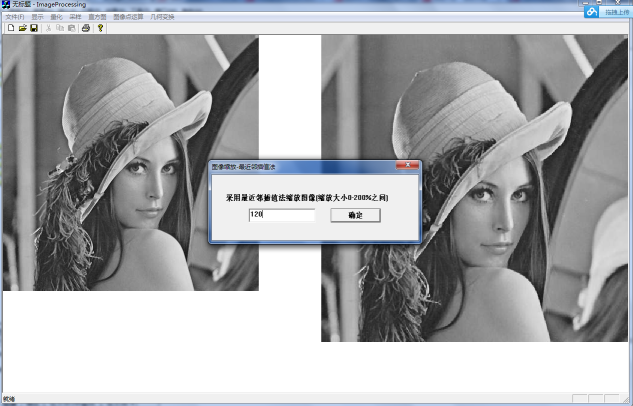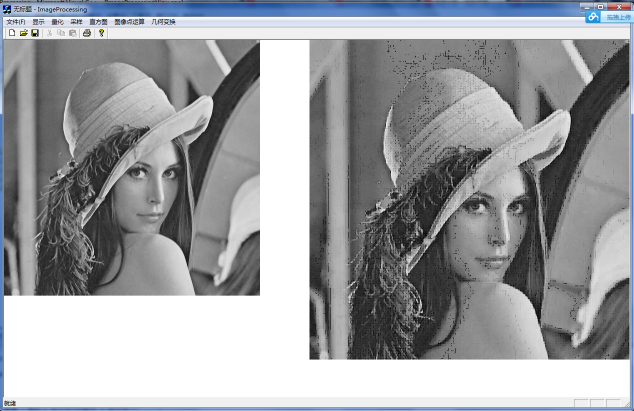但是同时当图片缩小是总是报错，图片缩放确实有点难，因为像素需要补齐4整数倍，同时需要修改消息头，同时像素矩阵的变换都非常复杂。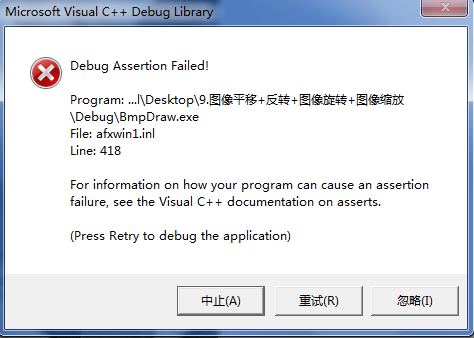最后还是希望文章对你有所帮助，如果文章有不足或错误之处，请海涵。自己给自己点个赞，挺不容易的，但还会继续写完~

（By:Eastmount 2015-06-04 下午5点   http://blog.csdn.net/eastmount/）


展开全文图像处理 几何变换
• Matlab:图像平移算法的原理实现，缺陷分析及优化 文章目录1.怎么样确定平移之后新的图片的模板呢?2.图像中的坐标平移转换是如何实现的呢？在实现平移算法之前，我们首先来了解平移算法的背景知识：那么我们如何将...
Matlab:图像平移算法的原理实现，缺陷分析及优化
文章目录1.怎么样确定平移之后新的图片的模板呢?2.图像中的坐标平移转换是如何实现的呢？在实现平移算法之前，我们首先来了解平移算法的背景知识：那么我们如何将图像坐标系转换为笛卡尔坐标系（也就是我们熟悉的数学坐标系）呢?3.上述步骤实现的图像平移算法的缺陷及优化问题一，当旋转角度过大时，无法实现图像平移?问题二，经过平移后的图像内部会存在大量黑点?问题三，如何更好地优化填充算法呢？
1.怎么样确定平移之后新的图片的模板呢?

这里，我们主要通过转换公式来实现：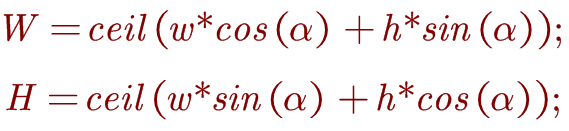W，H代表平移后的图片模块大小；而ceil()代表上取整，w，h代表原来图片的大小，Apha为旋转角度。经代码实现后，我们可以得到新的图片模板：
img=imread('pet.jpg');
subplot(2,2,1),imshow(img);
[h,w,k]=size(img);
a=80;
a=a*pi/180;
w_new=ceil(w*cos(a)+h*sin(a));
h_new=ceil(w*sin(a)+h*cos(a));
img_new=zeros(h_new,w_new,k);
%旋转
subplot(2,2,2),imshow(img_new);

如图所示：2.图像中的坐标平移转换是如何实现的呢？
在实现平移算法之前，我们首先来了解平移算法的背景知识：

以逆时针旋转为例：如图，旋转前的坐标为：
$x_0=rcos{\theta}$
$y_0=r\sin{\theta}$
而旋转后的坐标为：
$\chi_{1\ }:$
$=rcos{\left(\theta+\alpha\right)}$
$=rcos{\theta c o s{\alpha}}-rsin{\theta s i n{\alpha}}$
$=x_0cos{\alpha}-y_0sin{\alpha}$
$y_1:$
$=rsin{\left(\theta+\alpha\right)}$
$=r\sin{\theta c o s{\alpha}}+r\cos{\theta s i n{\alpha}}$
$=x_0\sin{\alpha}+y_0\cos{\alpha}$
通过以上的计算，我们可以得到平移矩阵：这个时候，如果你对图像处理有一定的了解的话，你会发现这里的坐标采用的是数学里面的笛卡尔坐标系，而并不是我们的图像的坐标系。所以，为了实现坐标的转换，我们首先得实现坐标系的统一。

那么我们如何将图像坐标系转换为笛卡尔坐标系（也就是我们熟悉的数学坐标系）呢?

坐标系平移：设图像宽度为W，高度为H，通过坐标系原点之间的位置关系，我们不难发现，由坐标系Ⅰ平移到Ⅱ的变换矩阵为：而其逆矩阵为：而我们得到图像平移的设计思路如下:

将像素所在的图像坐标系转换为笛卡尔坐标系。
将笛卡尔坐标系下的像素进行平移变换。
将像素所在的笛卡尔坐标系转换为图像坐标系。

coordination_shift=[1 0 -0.5*w;0 -1 0.5*h;0 0 1];%坐标系转换转换矩阵
rotation=[cos(a) -sin(a) 0; sin(a) cos(a) 0;0 0 1];%旋转矩阵
shift_back=[1 0 0.5*w_new;0 -1 0.5*h_new; 0 0 1];%还原坐标系矩阵

for y=1:h
for x=1:w
xy_position=coordination_shift*[x;y;1];%将像素所在的图像坐标系转换为笛卡尔坐标系。
xy_rotation=rotation*xy_position;%将笛卡尔坐标系下的像素进行平移变换。
xy_new=round(shift_back*xy_rotation);%将像素所在的笛卡尔坐标系转换为图像坐标系。
img_new(xy_new(2),xy_new(1),:)=img(y,x,:);
end
end
end
subplot(2,2,3),imshow(uint8(img_new));


通过如上的算法及代码实现，我们可以得到基于图像平移算法的实现操作。
3.上述步骤实现的图像平移算法的缺陷及优化
问题一，当旋转角度过大时，无法实现图像平移?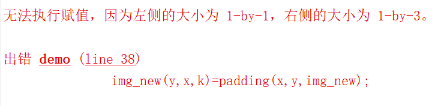原因分析：
当角度过大时，图像的像素位置经过笛卡尔坐标系下的旋转后，还原为图像坐标系后可能会发生越界情况，导致无法实现其平移。
解决方法：
当经过旋转后还原为图像坐标系的像素点发生越界情况后，舍弃这些像素点:
 xy_new=round(shift_back*xy_rotation);%将像素所在的笛卡尔坐标系转换为图像坐标系。
if xy_new(2)>0&&xy_new(2)<h+1&&xy_new(1)>0&&xy_new(1)<w+1
img_new(xy_new(2),xy_new(1),:)=img(y,x,:);


问题二，经过平移后的图像内部会存在大量黑点?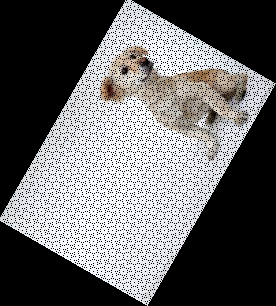原因分析：
因为旋转的计算采用了sin(),cos()三角函数计算，经过ceil()变化后，像素点会存在精度上的缺失。
解决方法：
这里，我采用的是当像素缺失点(x,y)的“四领域”均存在像素值时，该点填充像素点(x+1,y)的像素值。
for y=2:h_new-1
for x=2:w_new-1%这里需要注意的是，防止越界。
if img_new(y,x,:)==0
end
end
end
subplot(2,2,4),imshow(uint8(img_new));

%自定义判定函数
result=0;
if img(y-1,x,:)>0
if img(y,x-1,:)>0
if img(y+1,x,:)>0
if img(y,x+1,:)>0
result=img(y,x+1,:);
else
result=0;
end
end
end
end
end

效果展示：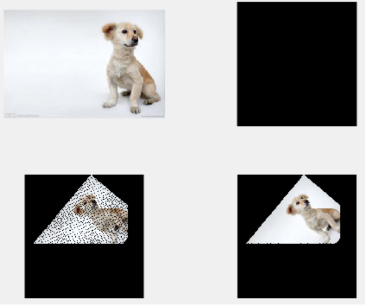问题三，如何更好地优化填充算法呢？

其实经过上述的填充算法，当旋转角度小于90°时，填充的效果较好；当旋转角度大于90时，如何更好地优化填充算法呢?这是留给笔者和阅读这篇文章的读者值得思考的问题。(｡◕ˇ∀ˇ◕)


展开全文算法 opencv 计算机视觉
• 介绍 坐标变换矩阵是一个3*3的矩阵，用来对图形进行坐标变化，将原来的坐标点转移到新的坐标点，因为一个图片是有点...平移旋转 绕原点逆时针旋转θ度角的变换公式是 x’ = xcosθ − ysinθ 与 y。’ = xsinθ + ycos图像处理 缩放 变换
• 最近刚开始学图像处理，因此写下记录下来。  图像平移原理 src=imread('Fig3.tif'); [m,n]=size(src); des=zeros(m,n); left=[1,0,-50;0,1,-50;0,0,1];//向左上方向移动50 for i=1:m for j=1:n t=left*[i;j;1];...
• 本文主要讲述基于VC++6.0 MFC图像处理的应用知识，主要结合自己大三所学课程《数字图像处理》及课件进行讲解，主要通过MFC单文档视图实现显示BMP图片空间几何变换，包括图像平移、图形旋转、图像反转倒置镜像和图像...数字图像处理
• 图像平移并不会影响图像的频谱，同时，图像的相位会随着图像的旋转而旋转。   Part I 平移和旋转对频谱的影响 下面我用矩形的频谱图来说明图像中矩形的平移并不会对频谱有丝毫的影响。   Matlab代码：...图像处理
• 图像的一些操作，可以通过矩阵进行简化，例如，旋转，平移，缩放数字图像处理
• 形态学图像处理的基本理论是数学形态学（Mathematical morphology）。数学形态学的语言是集合论，集合表示图像中的对象。 在形态学中，集合的反射和平移广泛用来表达基于 结构元（SE） 的操作：研究一幅图像中感兴趣...形态学图像处理
• 本文主要讲述基于VC++6.0 MFC图像处理的应用知识，主要结合自己大三所学课程《数字图像处理》及课件进行解说，主要通过MFC单文档视图实现显示BMP图片空间几何变换。包含图像平移、图形旋转、图像反转倒置镜像和图像...
• 有的时候变换可能比较复杂，比如平移之后又旋转，旋转之后又平移，又缩放。 直接用公式计算，不但复杂，而且效率低下。这时可以借助变换矩阵和矩阵乘法，将多个变换合成一个。 最后只要用一个矩阵对每个点做一次...
• 本文为参考这位...代数运算对多幅图像处理，也不改变像素的空间位置；几何运算对单幅图像处理，改变像素的空间位置，几何运算包括两个独立的算法：空间变换算法和灰度级插值算法...
• 对于一维函数：未平移前，FT的一个周期是沿原点对称分布的。讨论DFT，假设频率范围为[0，1，……，M－1，M]，则DFT的频谱分布在原点0的两侧，左：[M/2，M/2-1，……，0]，右侧[0，……，M/2-1，M/2] 同样，将上述...图像处理
• 基本图像处理 缩放scale 旋转rotate 裁剪crop 填充pad 平移translate 翻转flip 图像的仿射变换Affine transformation基本图像处理1. 缩放scale缩放通过cv2.resize()实现函数说明：cv2.resize(src, dsize[, dst[, fx...图像处理 opencv python
• 遇到了一些情况需要将图片...仿射变换可以通过一系列的原子变换的复合来实现，包括平移，缩放，翻转，旋转和剪切。 1）图像的几何变换 对图像进行放大、缩小、旋转等操作，会改变原图中各区域的空间关系，这类操...
• 图像的hu矩是一种具有平移、旋转和尺度不变性的图像特征。 普通矩的计算： f(x,y)的p+q阶原点矩可以表示为： 而数字图像是一个二维的离散信号，对上述公式进行离散化之后： 其中C与R分别表示图像的列与行。各阶...图像处理 尺度不变性
• 一、图像的放大和缩小原理 这里的放大和缩小不是指在物理空间中某一个物体的放大和缩小。二维空间坐标(x,y)以(0, 0)为中心在水平方向上缩放Sx倍，在垂直方向上缩放Sy倍，指的是变换后坐标位置距离(0,0)的水平距离...
• 1.概念词语1）图像的几何变换 对图像进行放大、缩小、旋转等操作，会改变原图中各区域的空间关系，这类操作就是图像的几何变换。2）仿射变换 对原来的x和y坐标分别进行线性的几何变换，得到新的x和y，这种变换就是...
...